ICSE Class 10 Maths Sample Question Paper – Mock 1 with Solutions are provided at BYJU’S that can be accessed at any time online as well as in pdf which can be downloaded easily. Though there is no particular textbook for the ICSE maths class 10 our experts have given a list of books that will help the students to cover the syllabus class 10 maths ICSE.

ICSE Maths requires a lot of practice to score full marks for all the questions that appear in the exam. Practising sample papers for class 10 maths of ICSE will help the students in boosting their analytical and problem-solving skills.

### ICSE Class 10 Maths Mock Sample Paper 1 with Solutions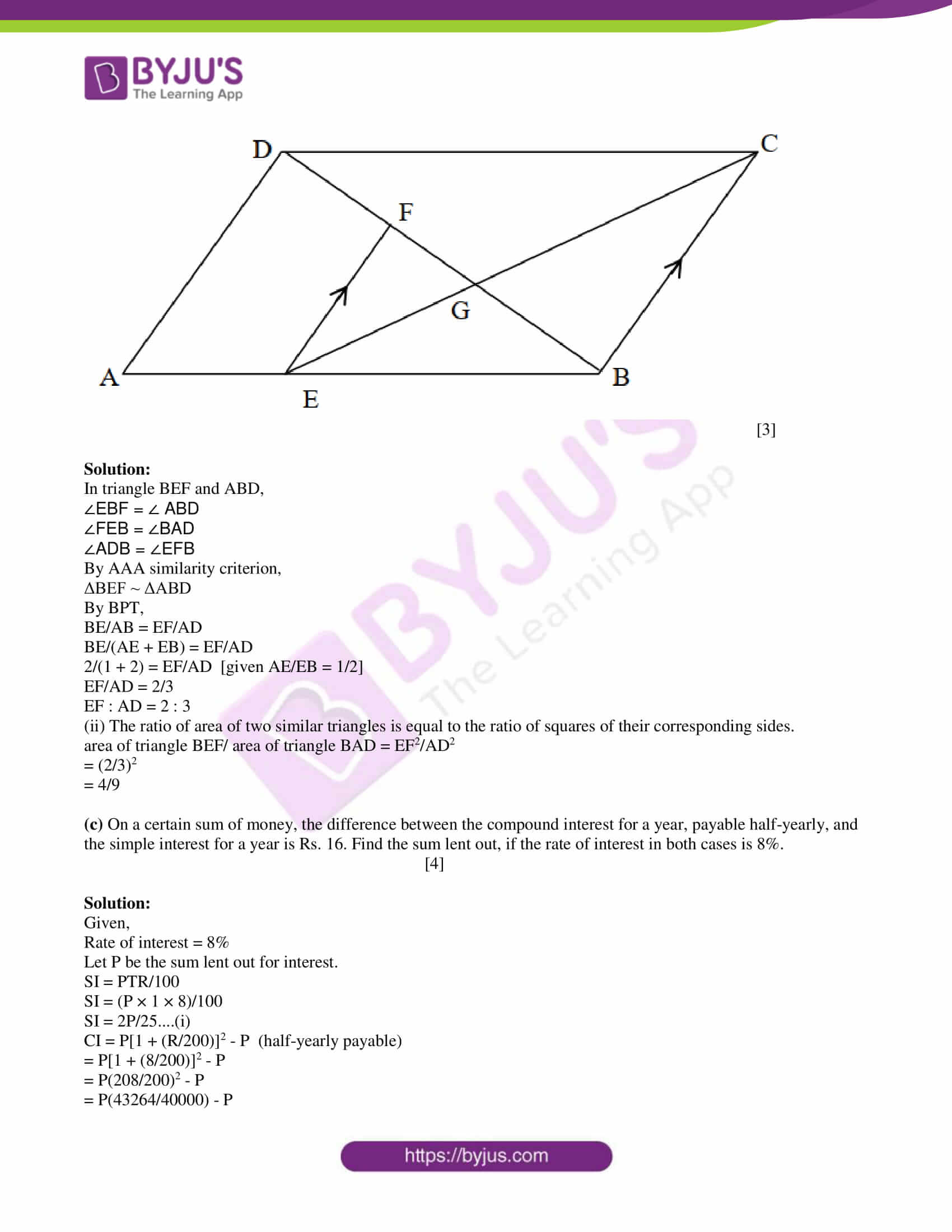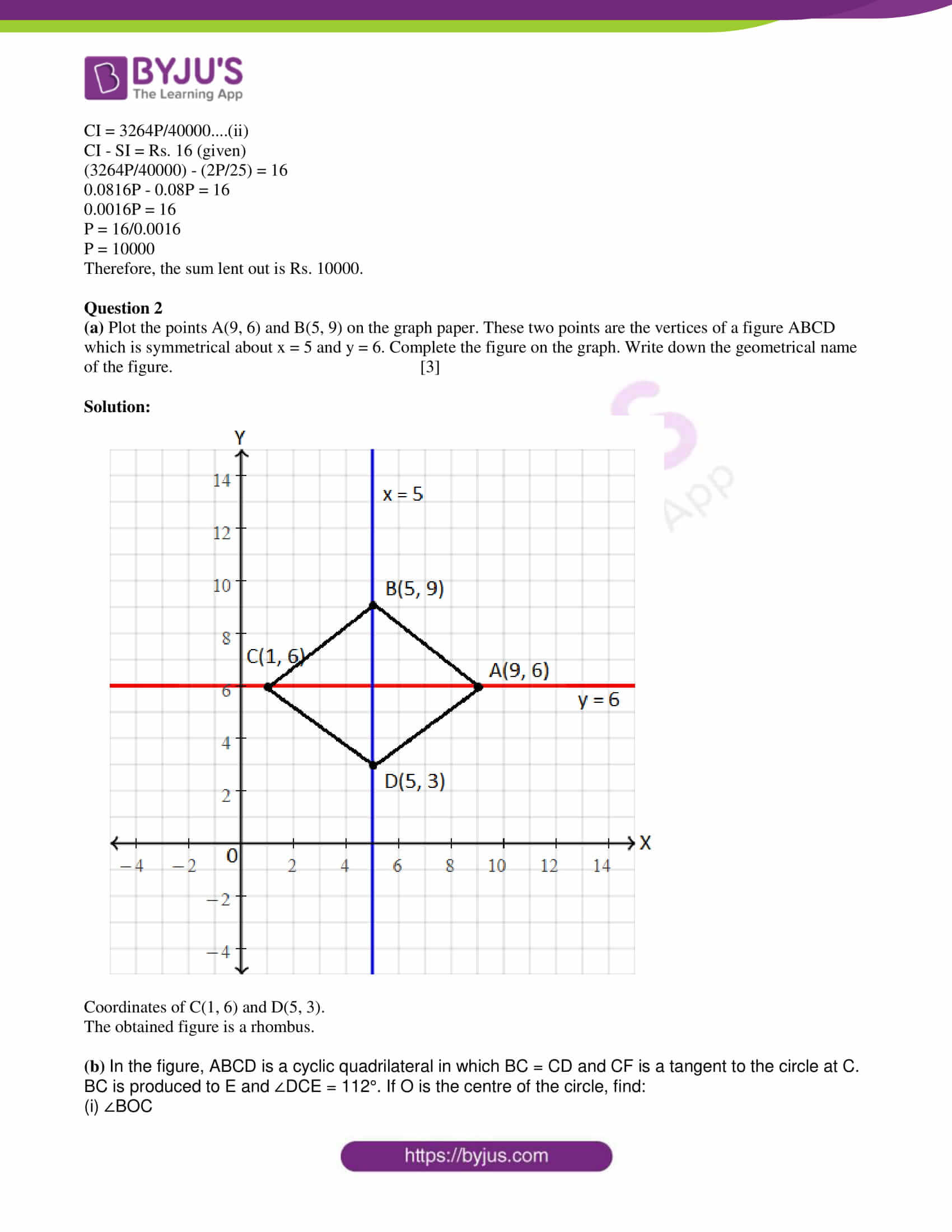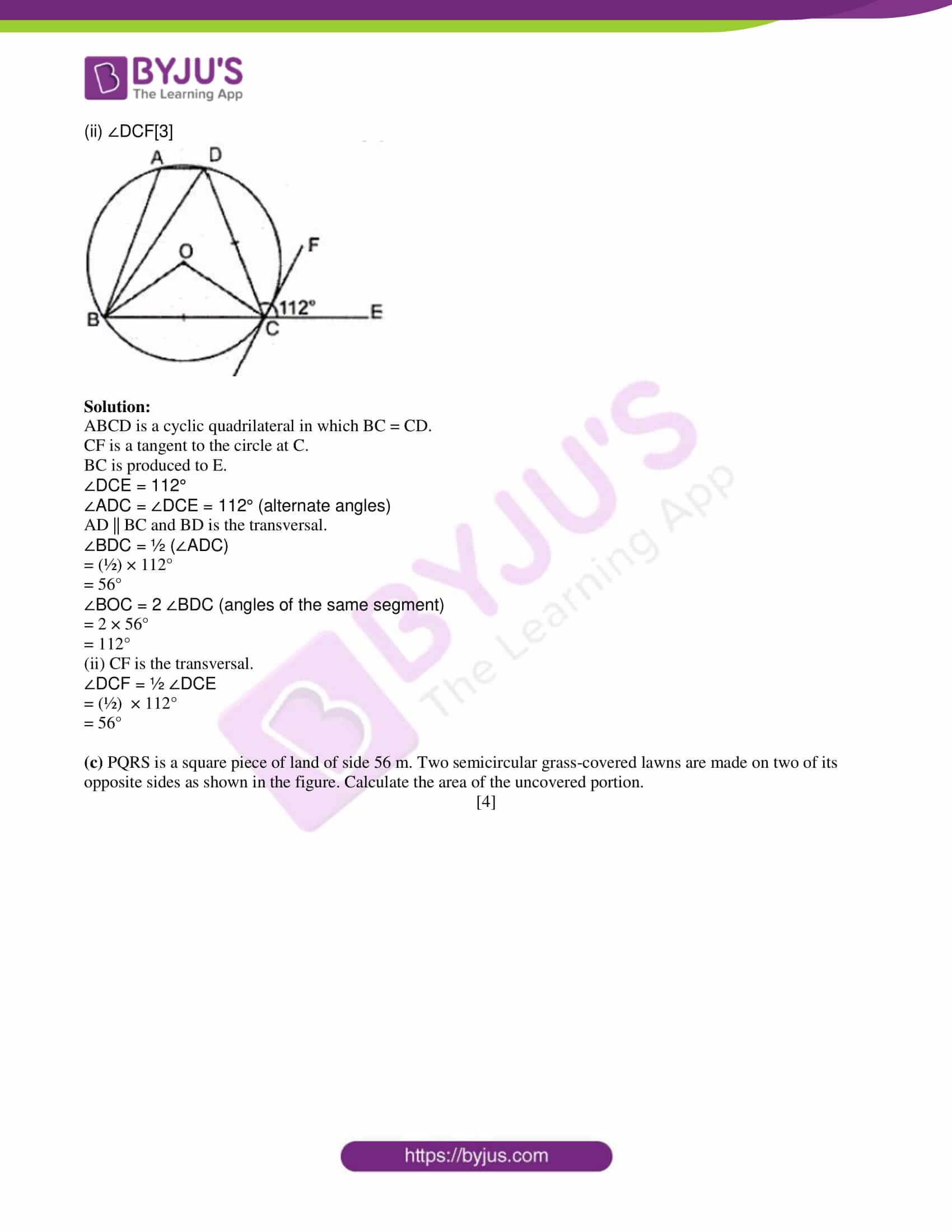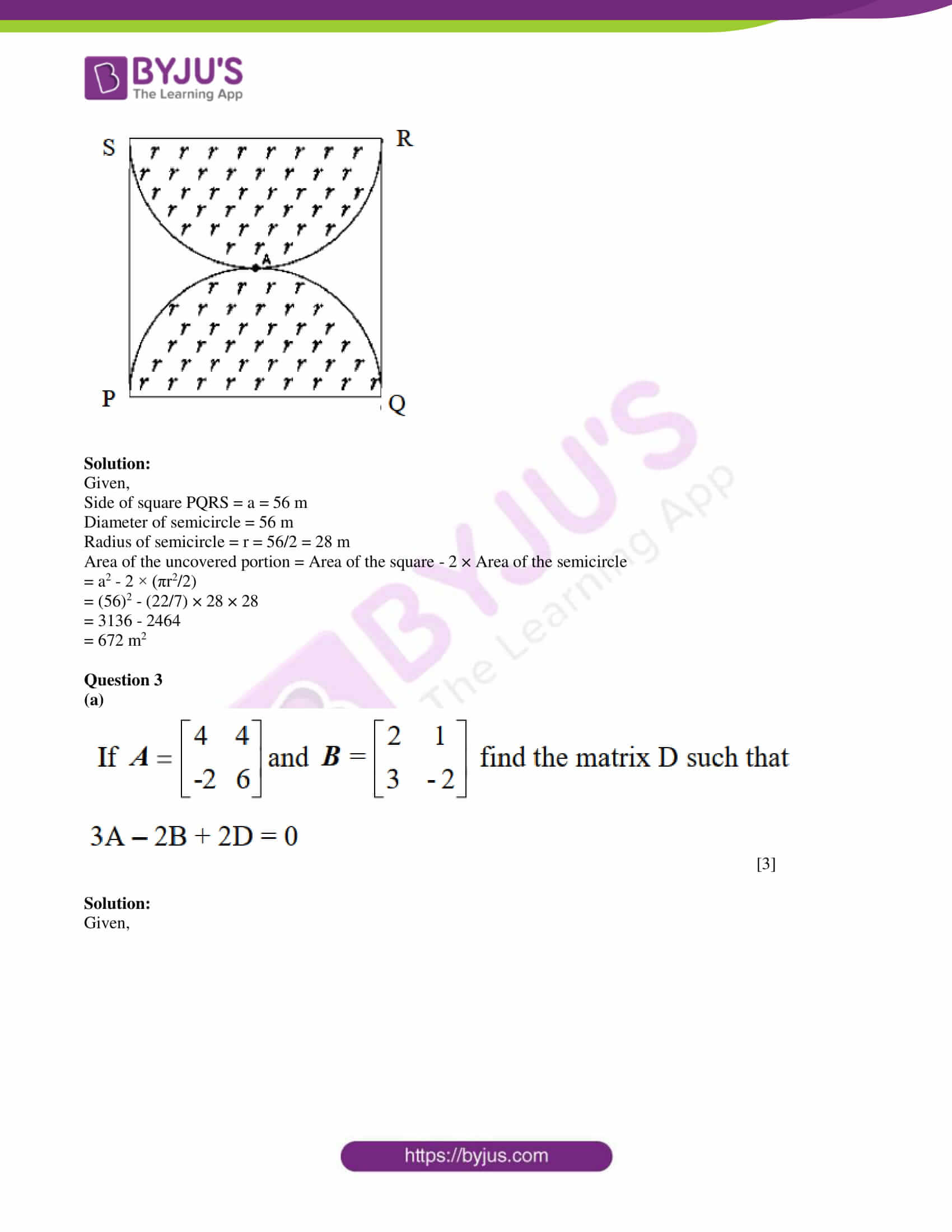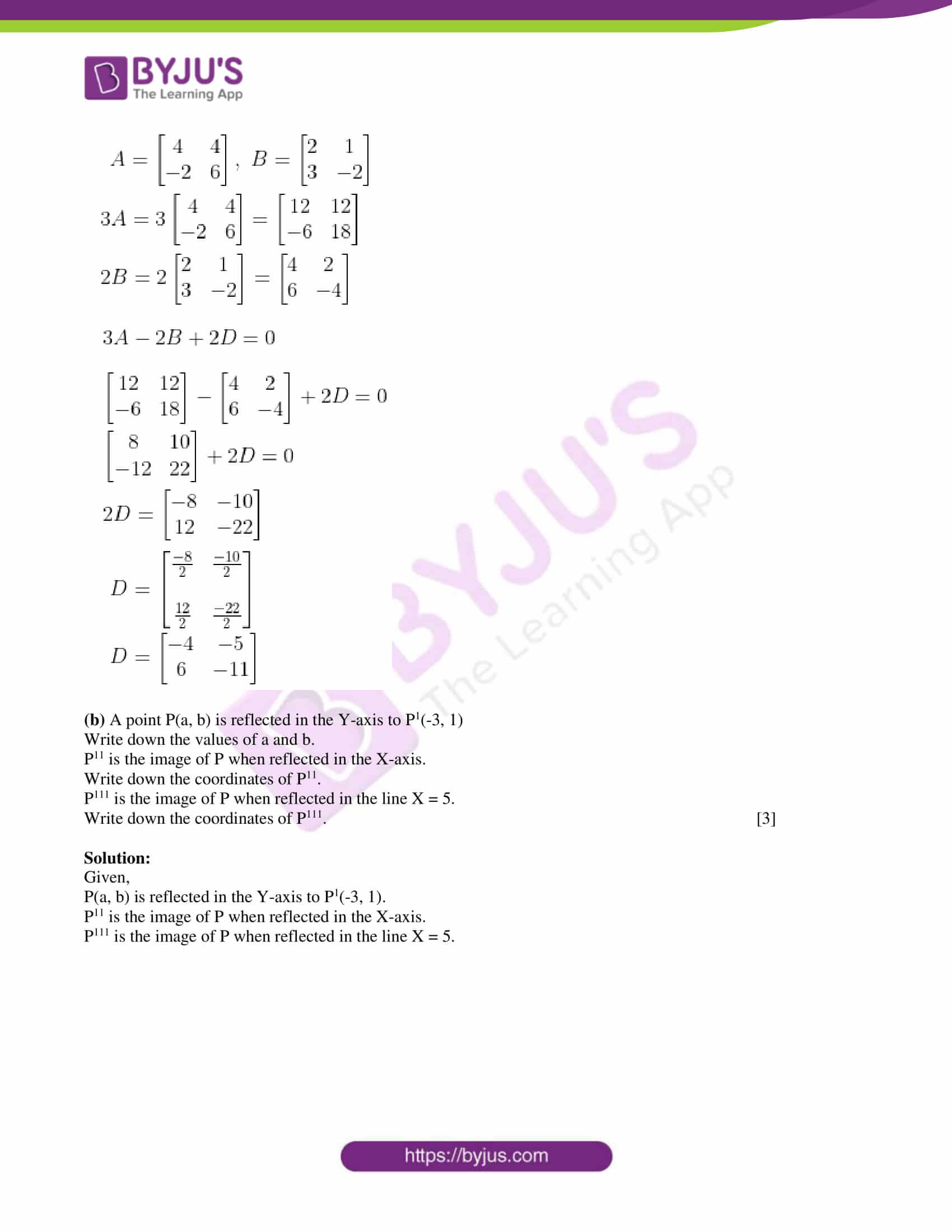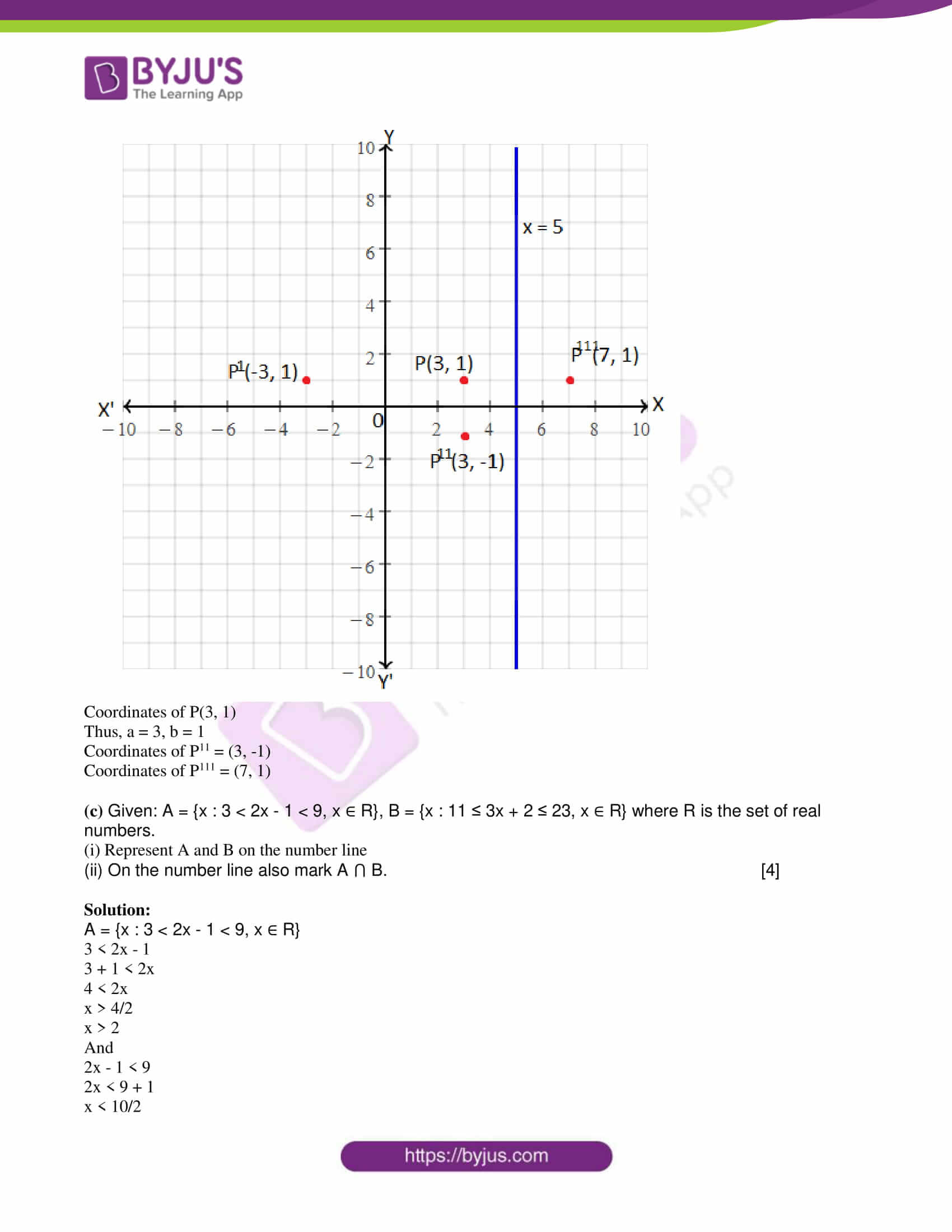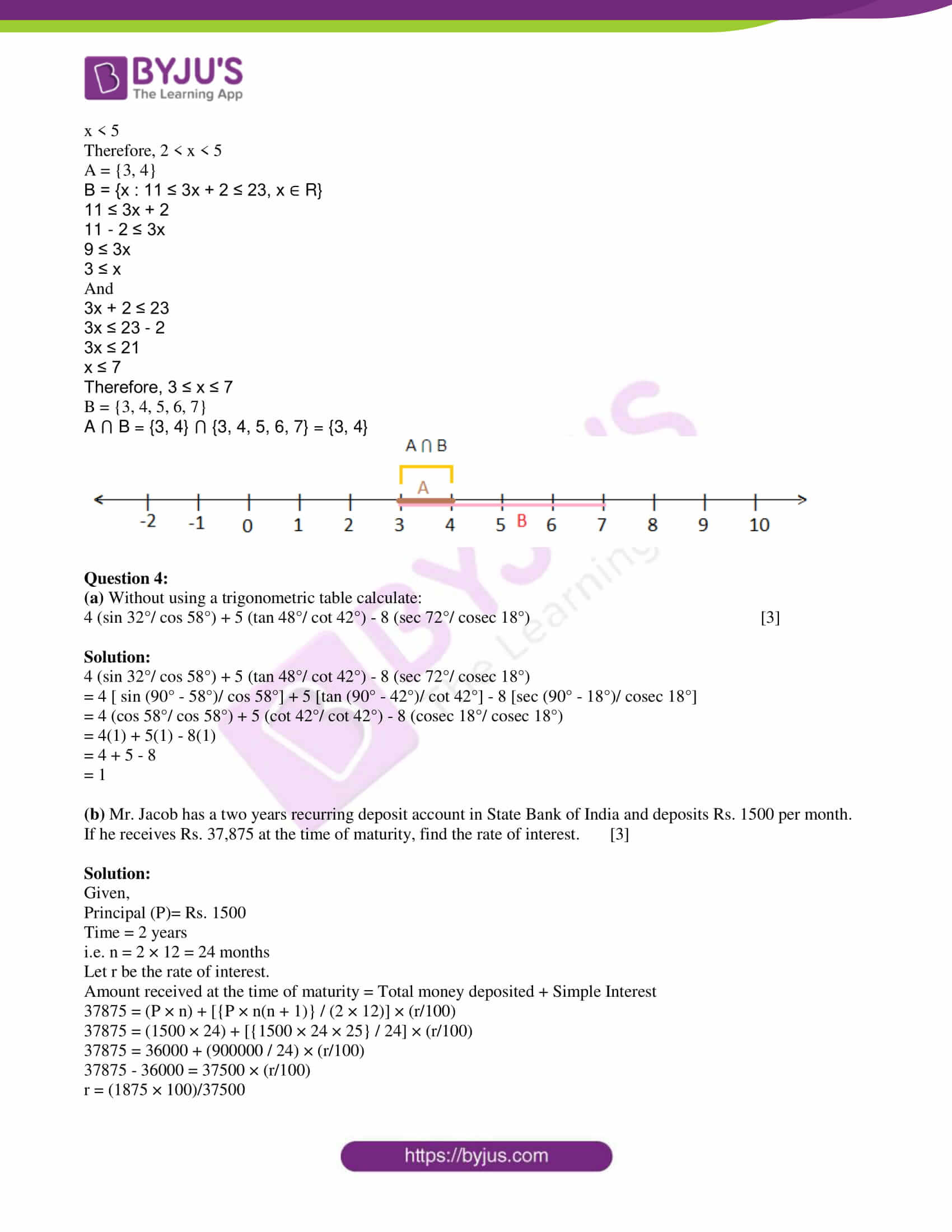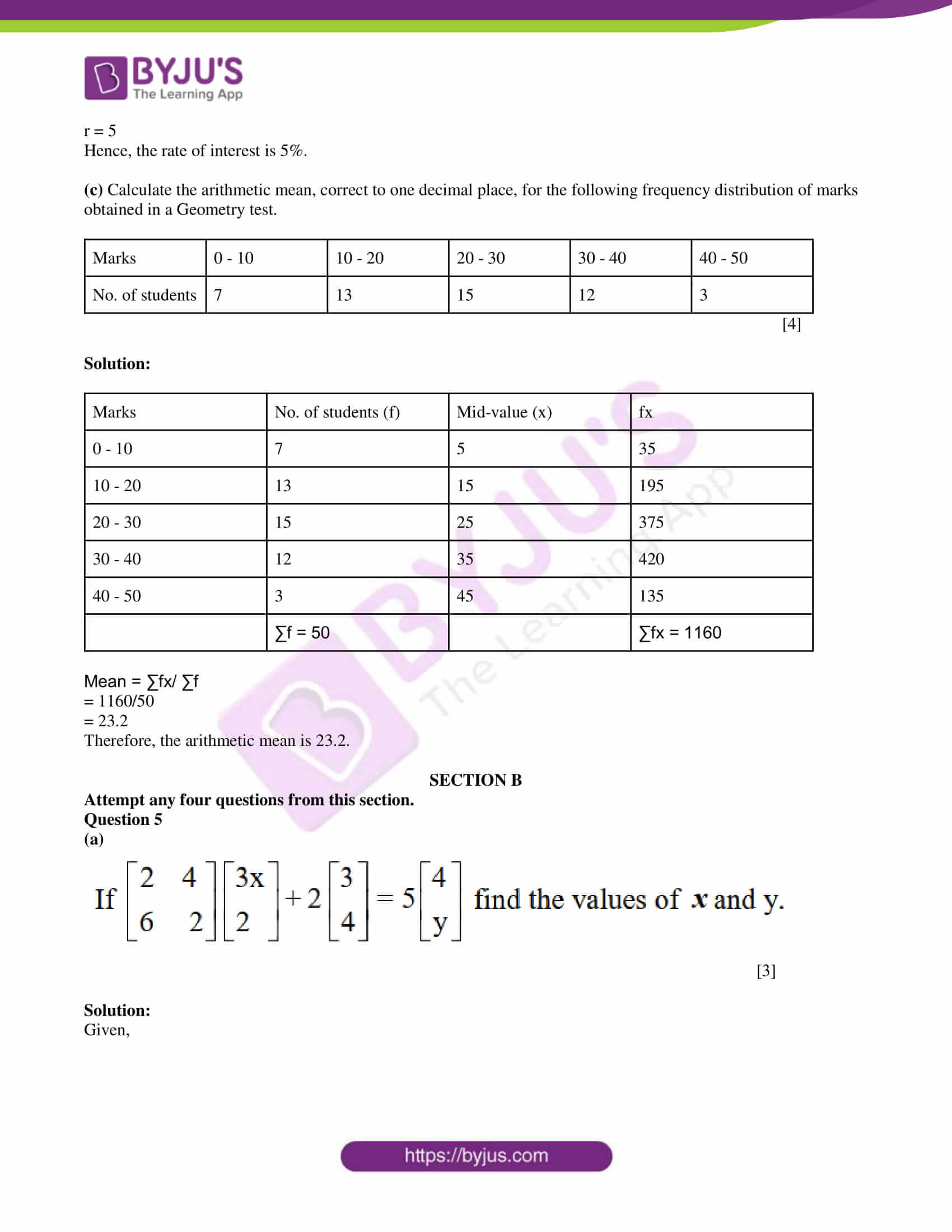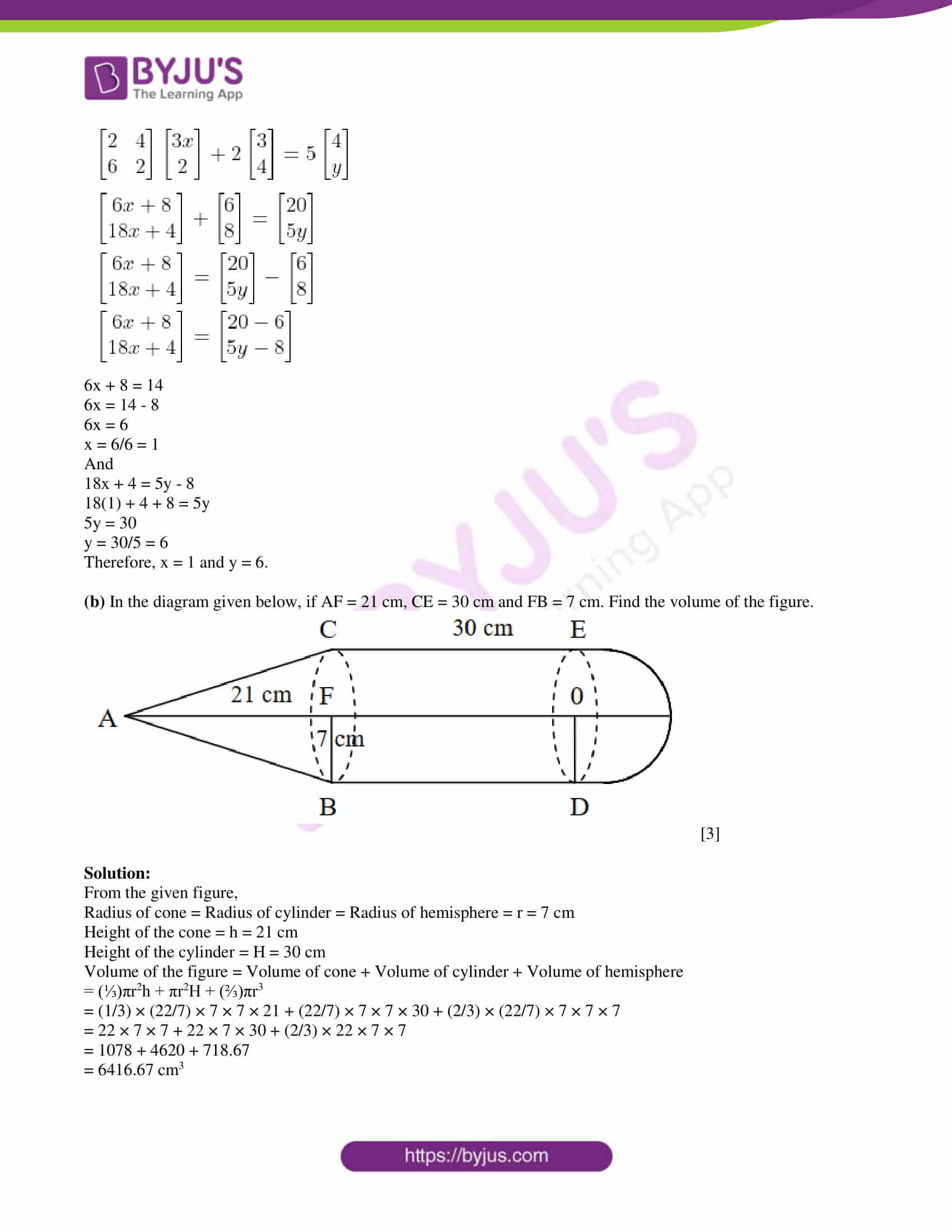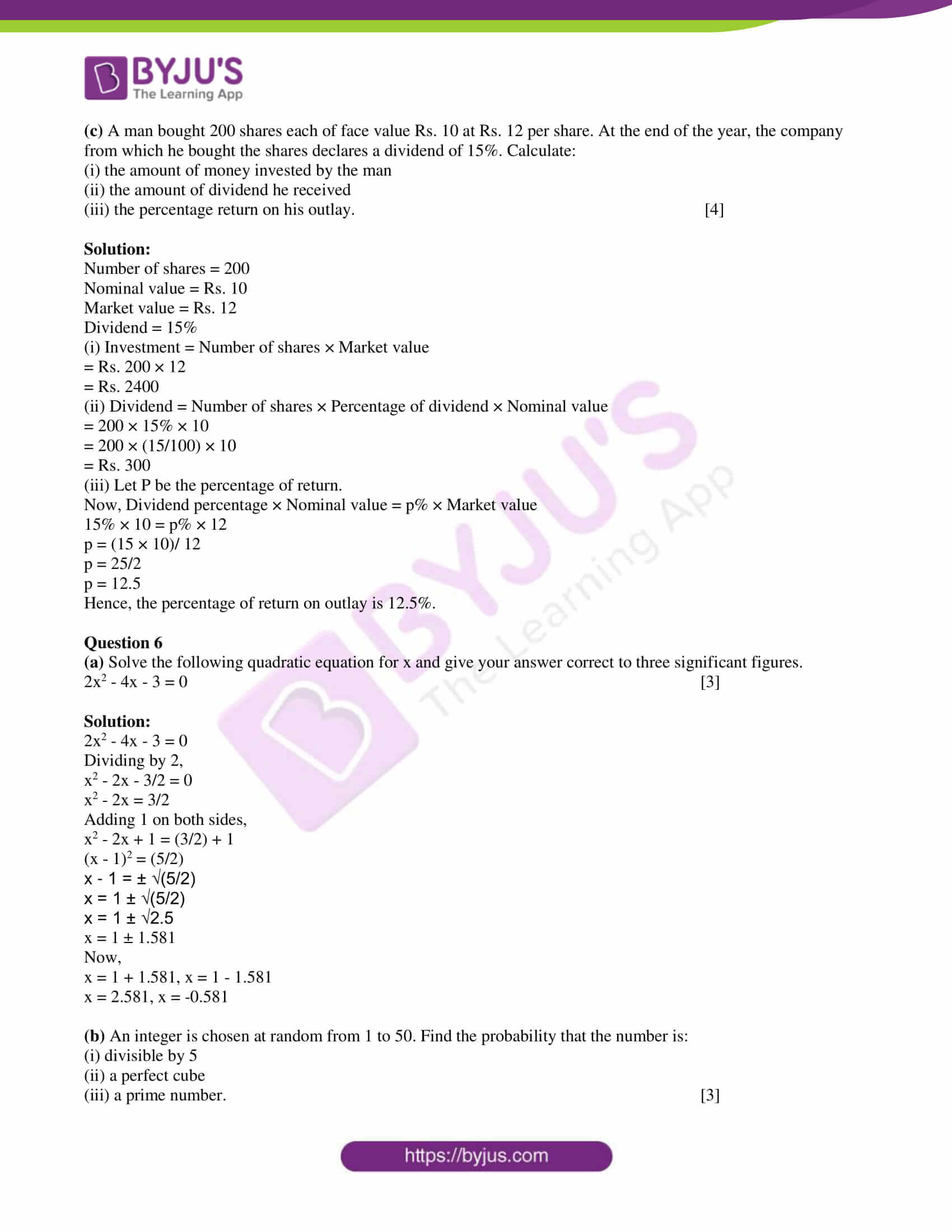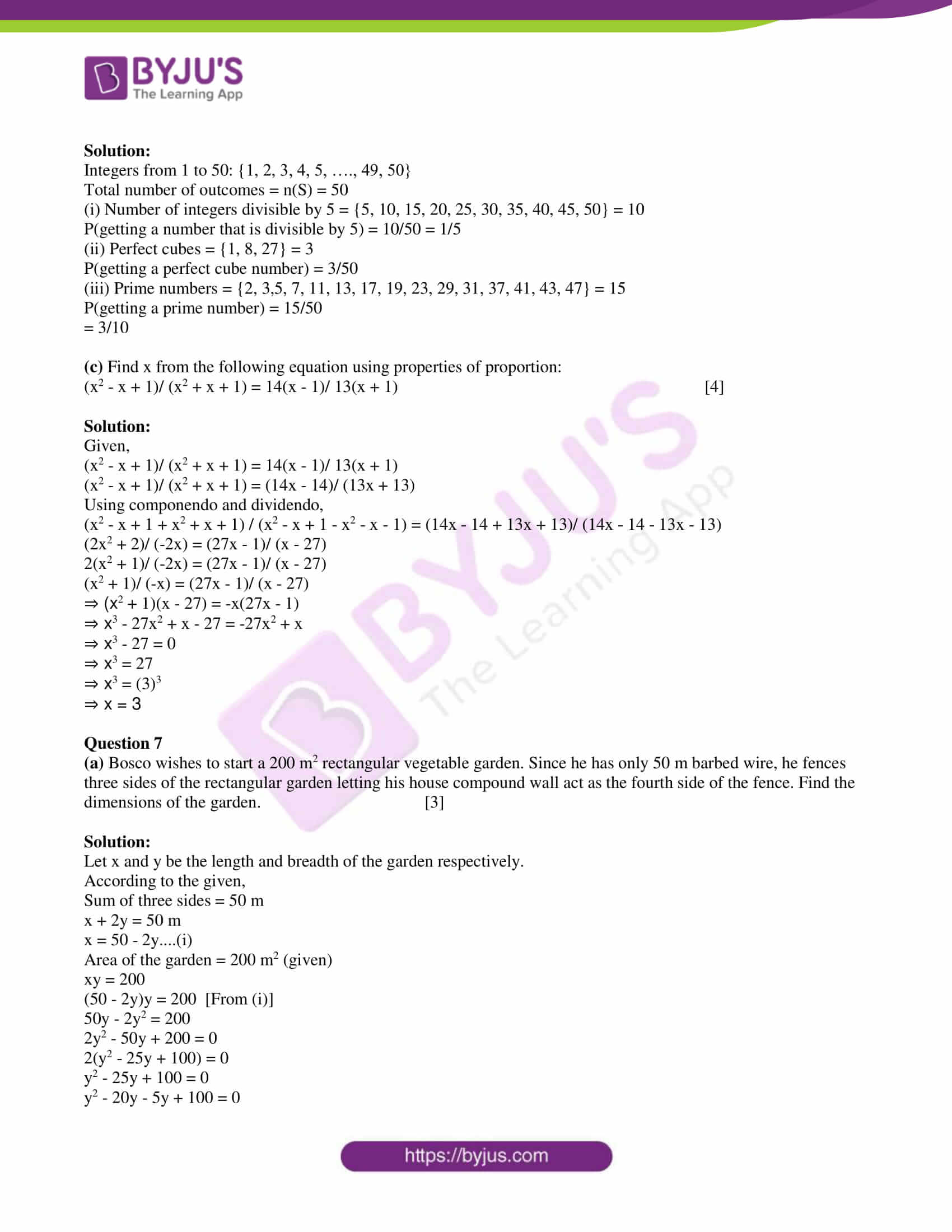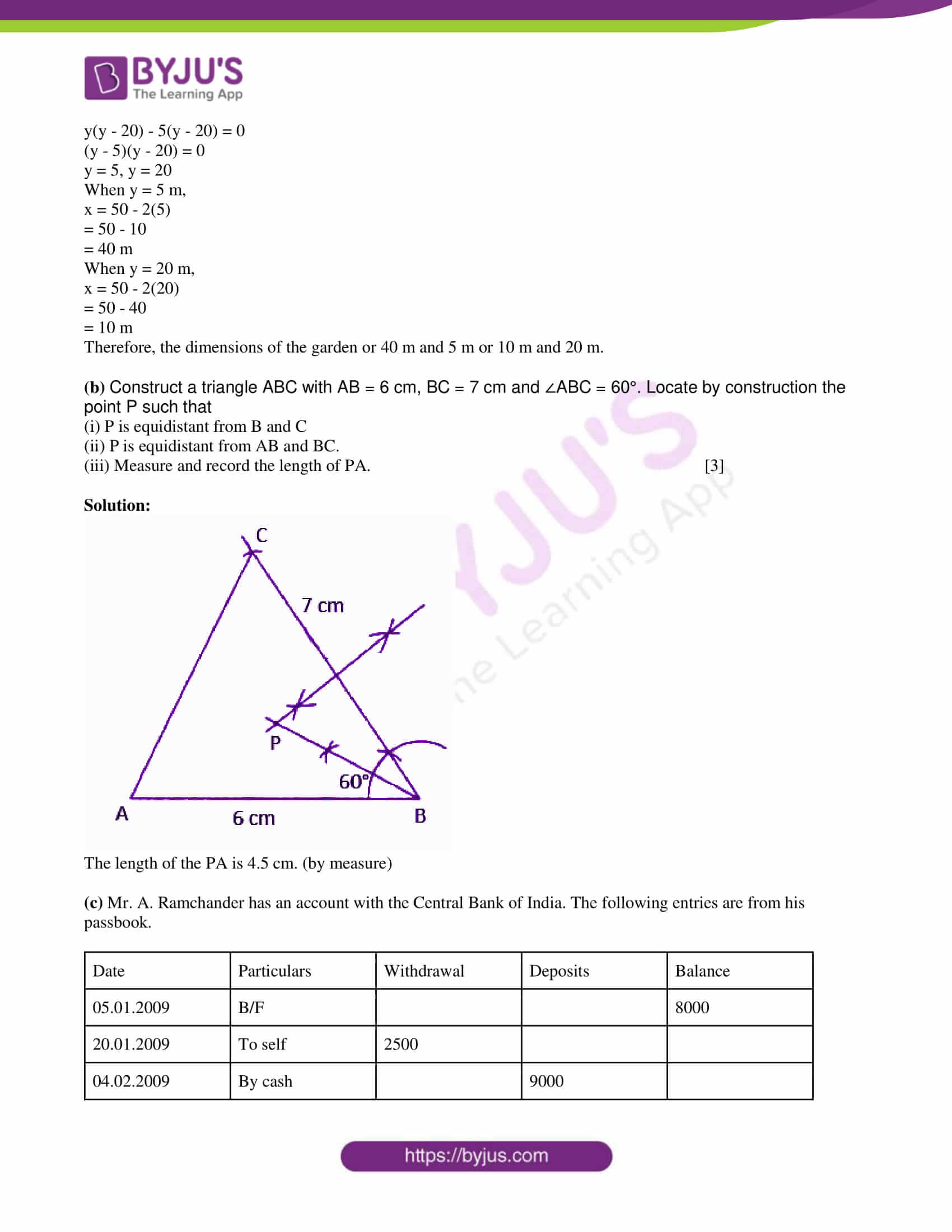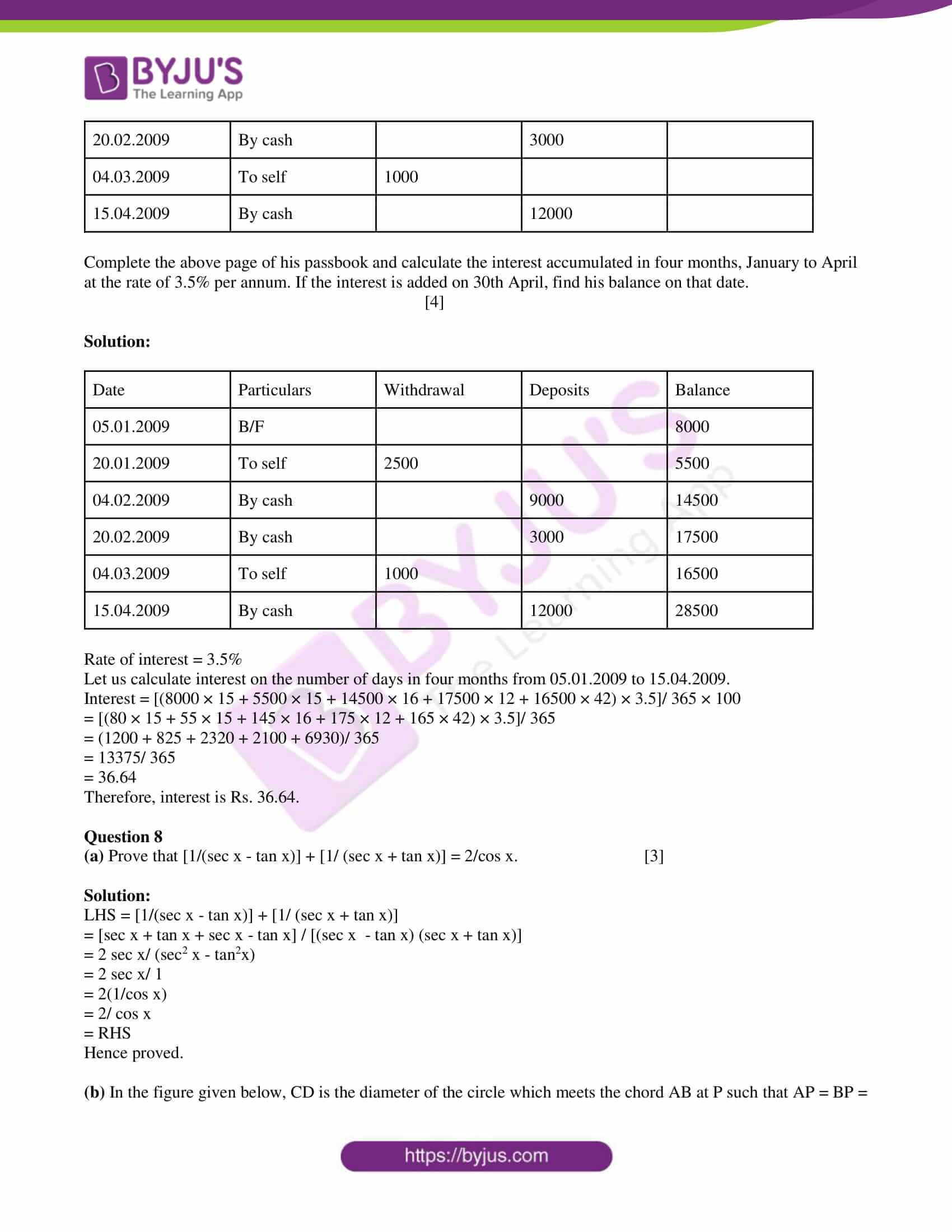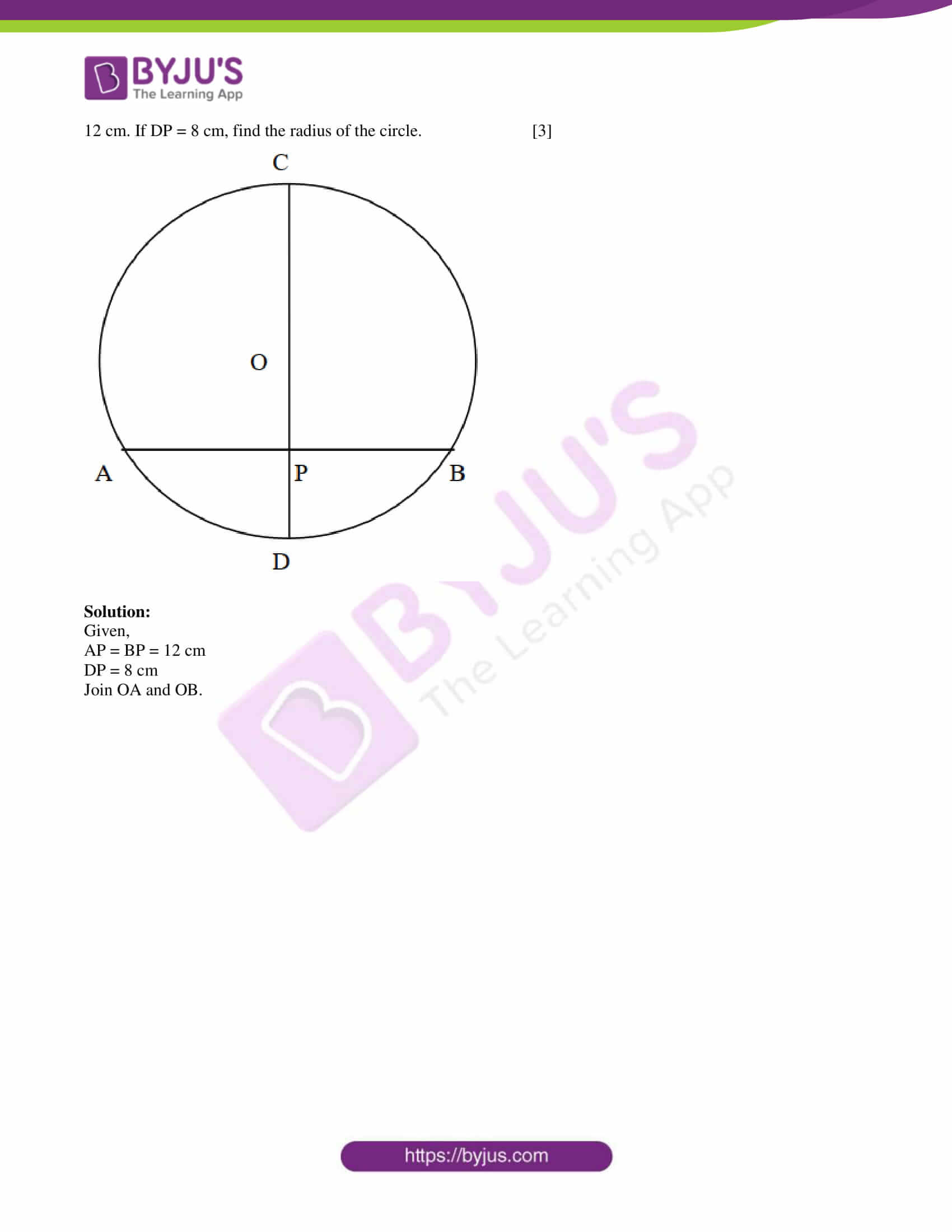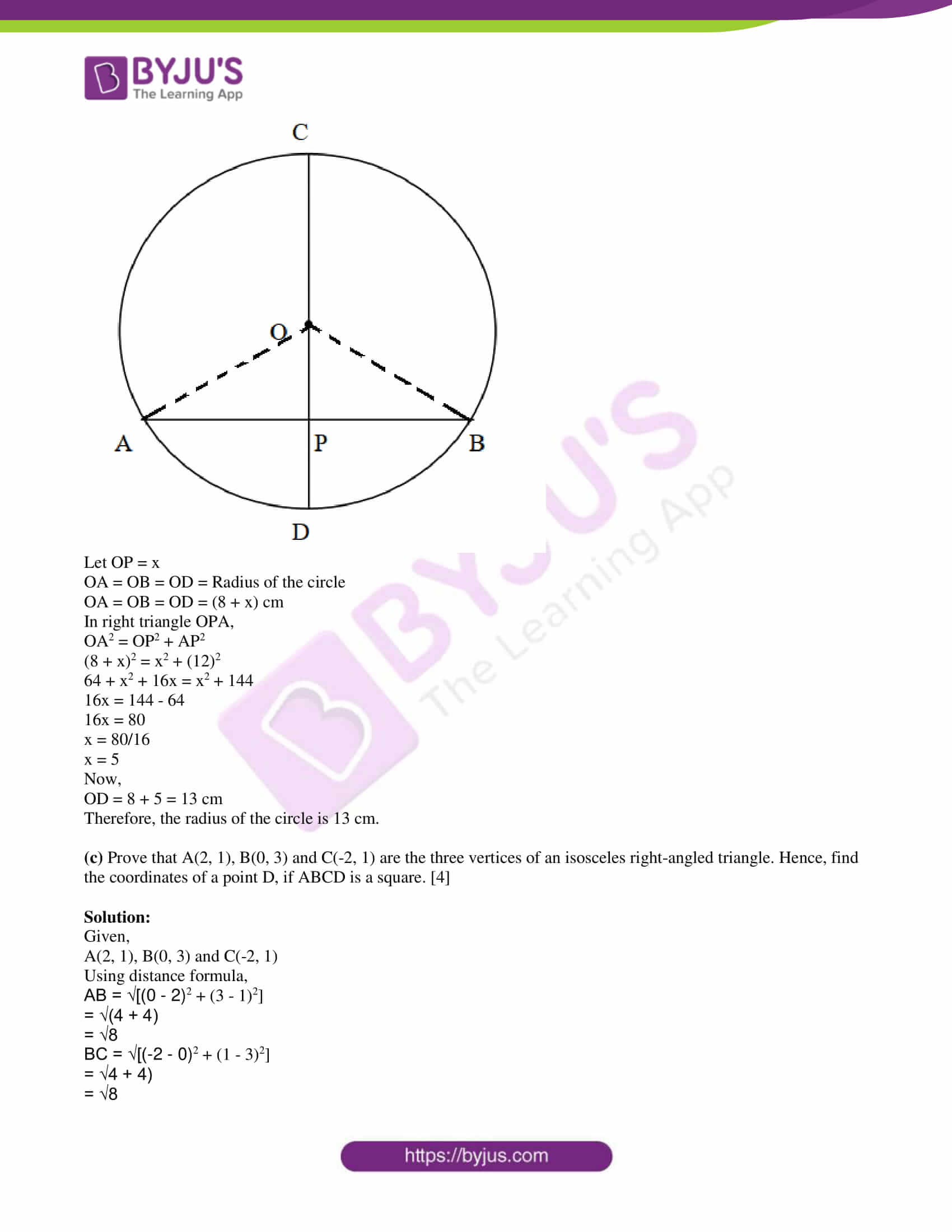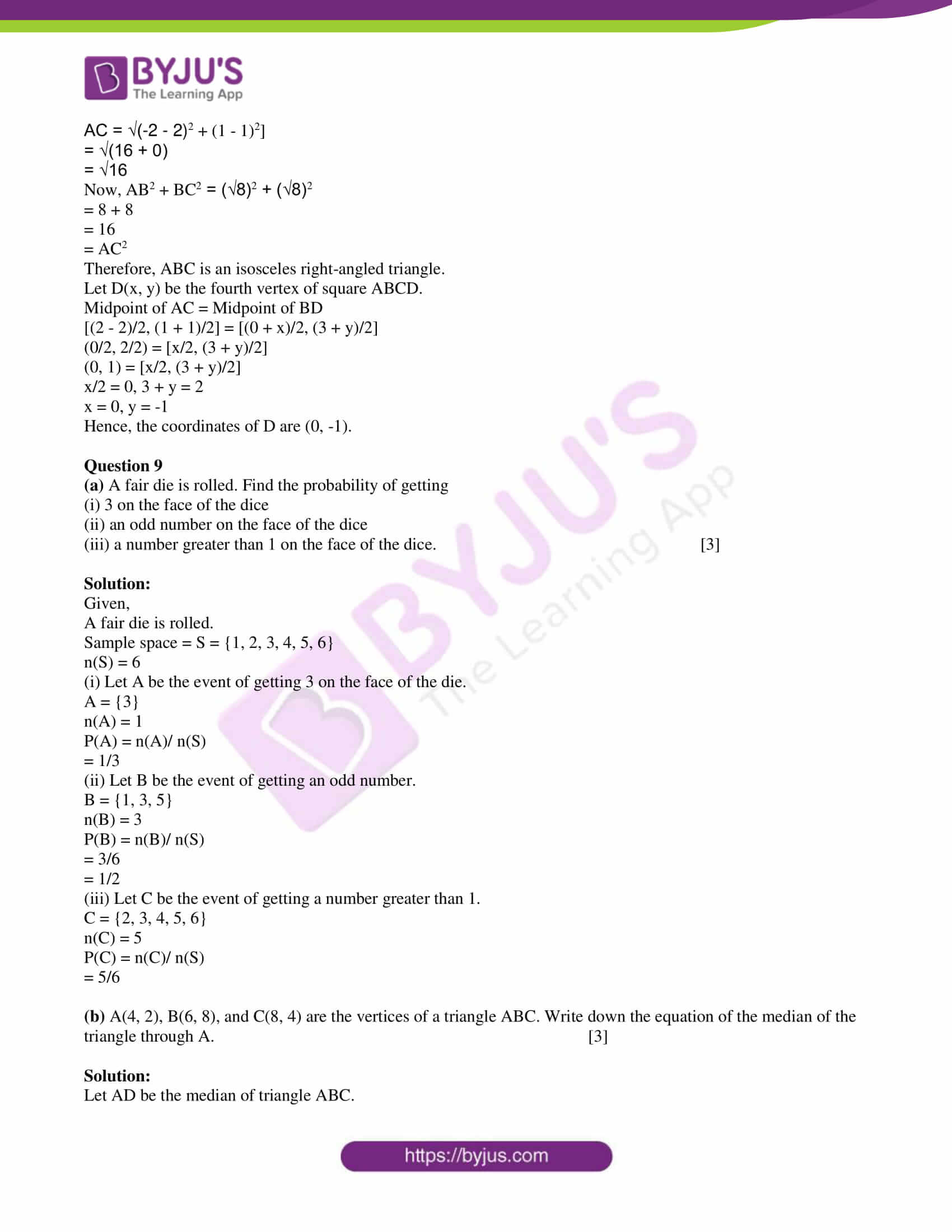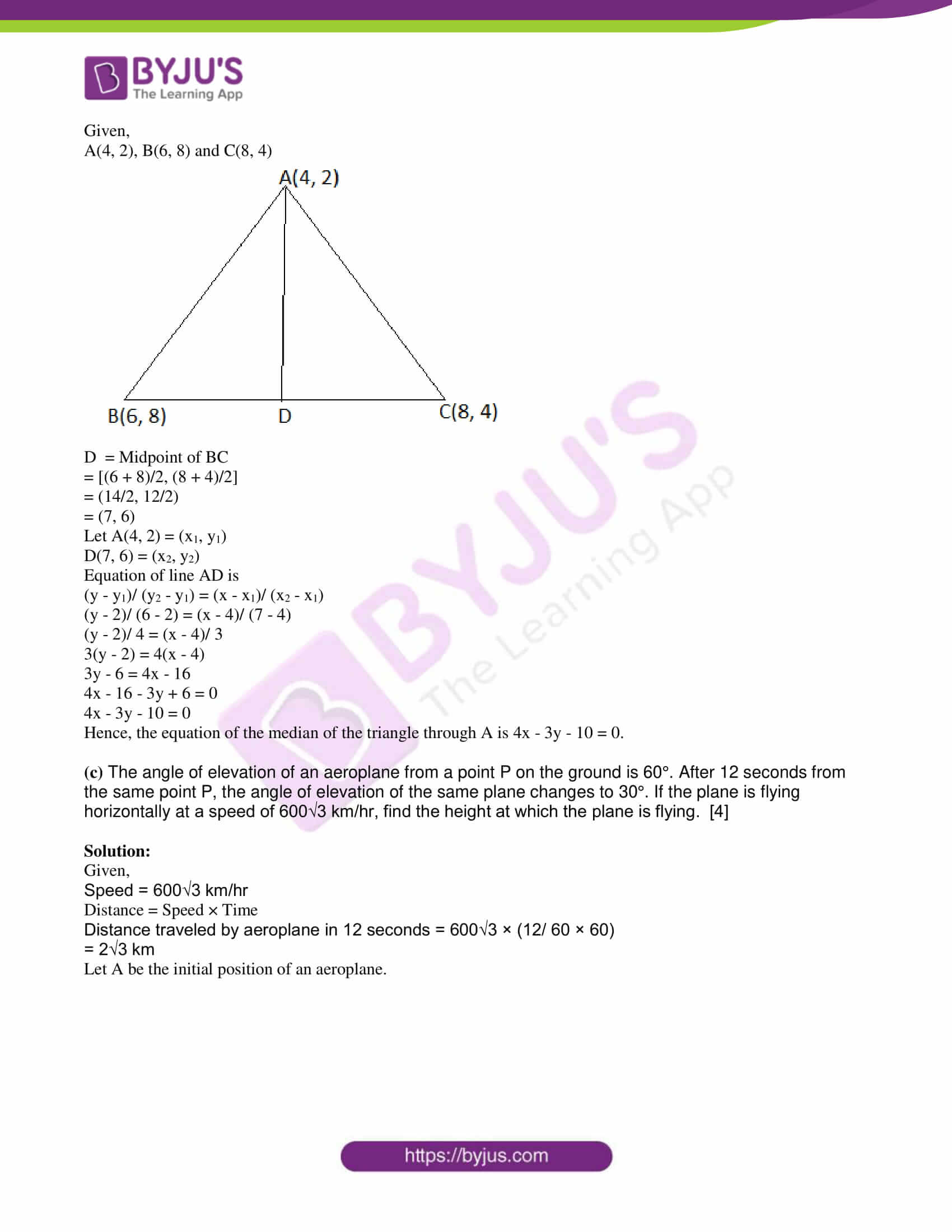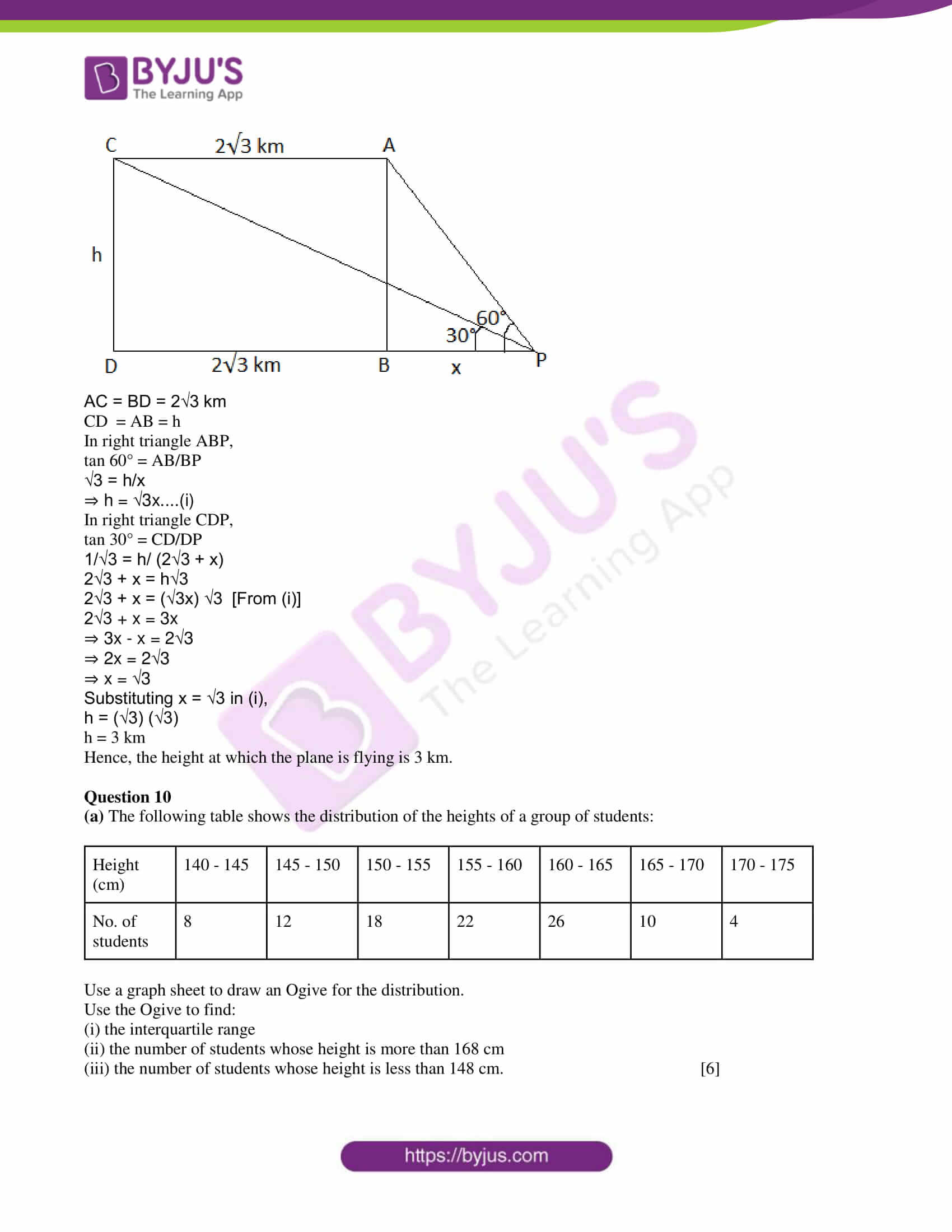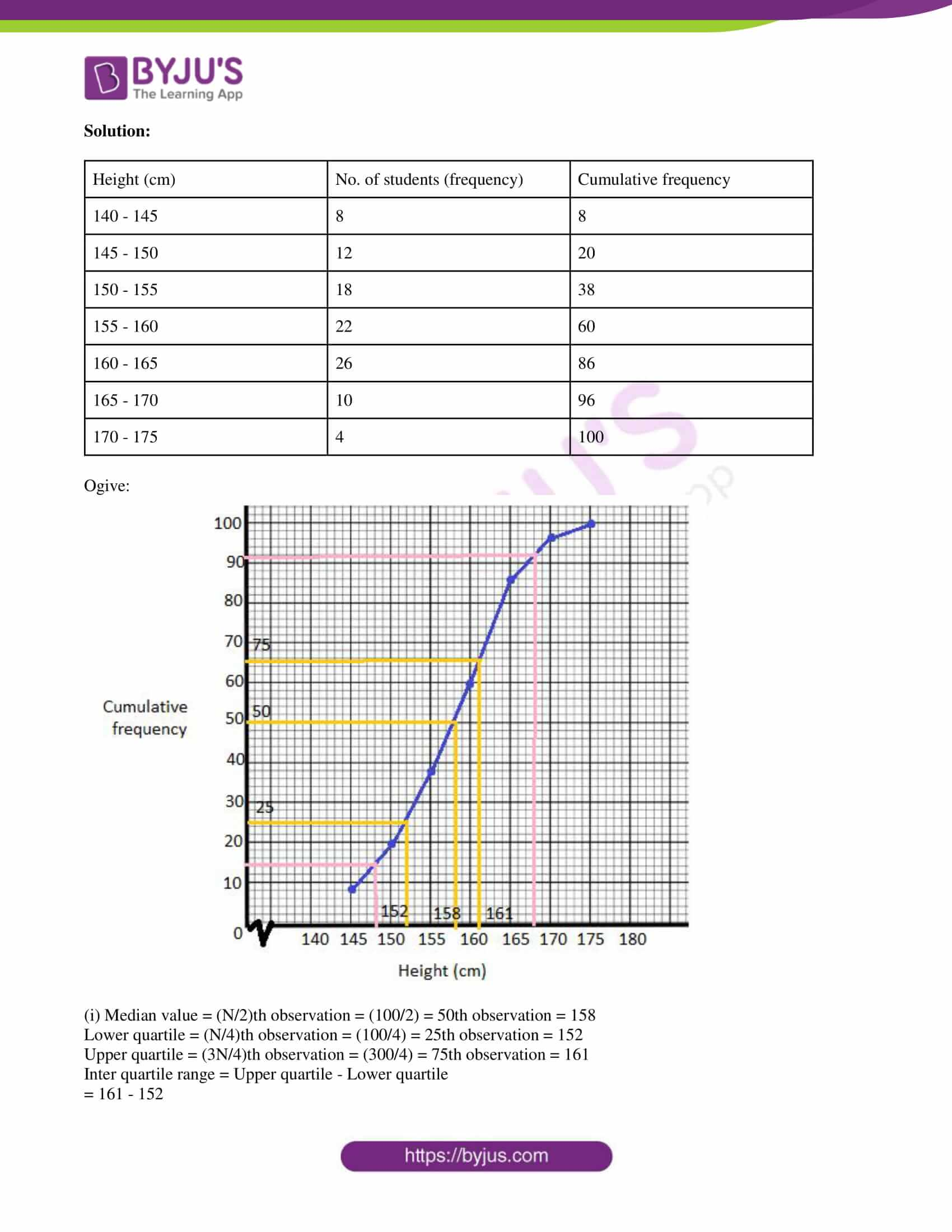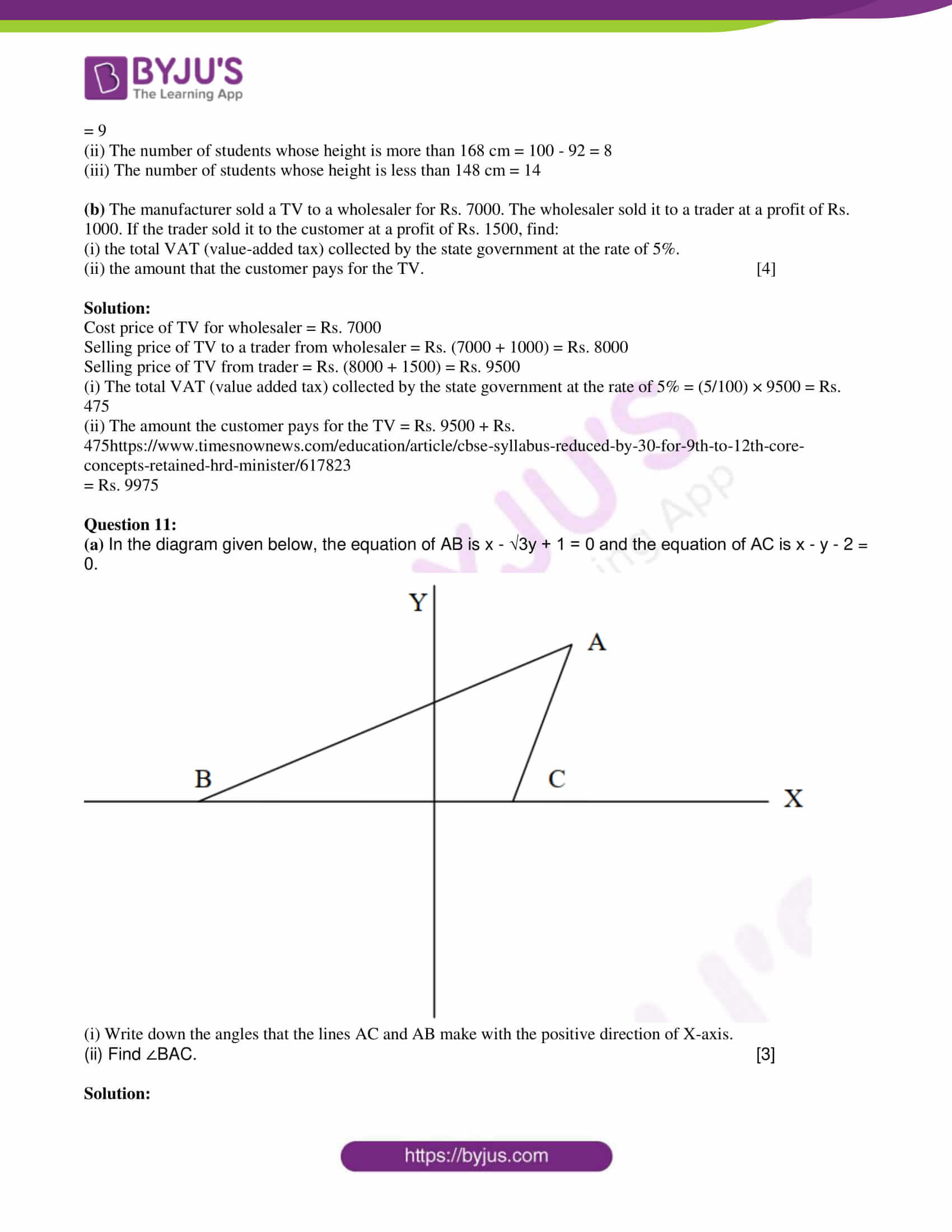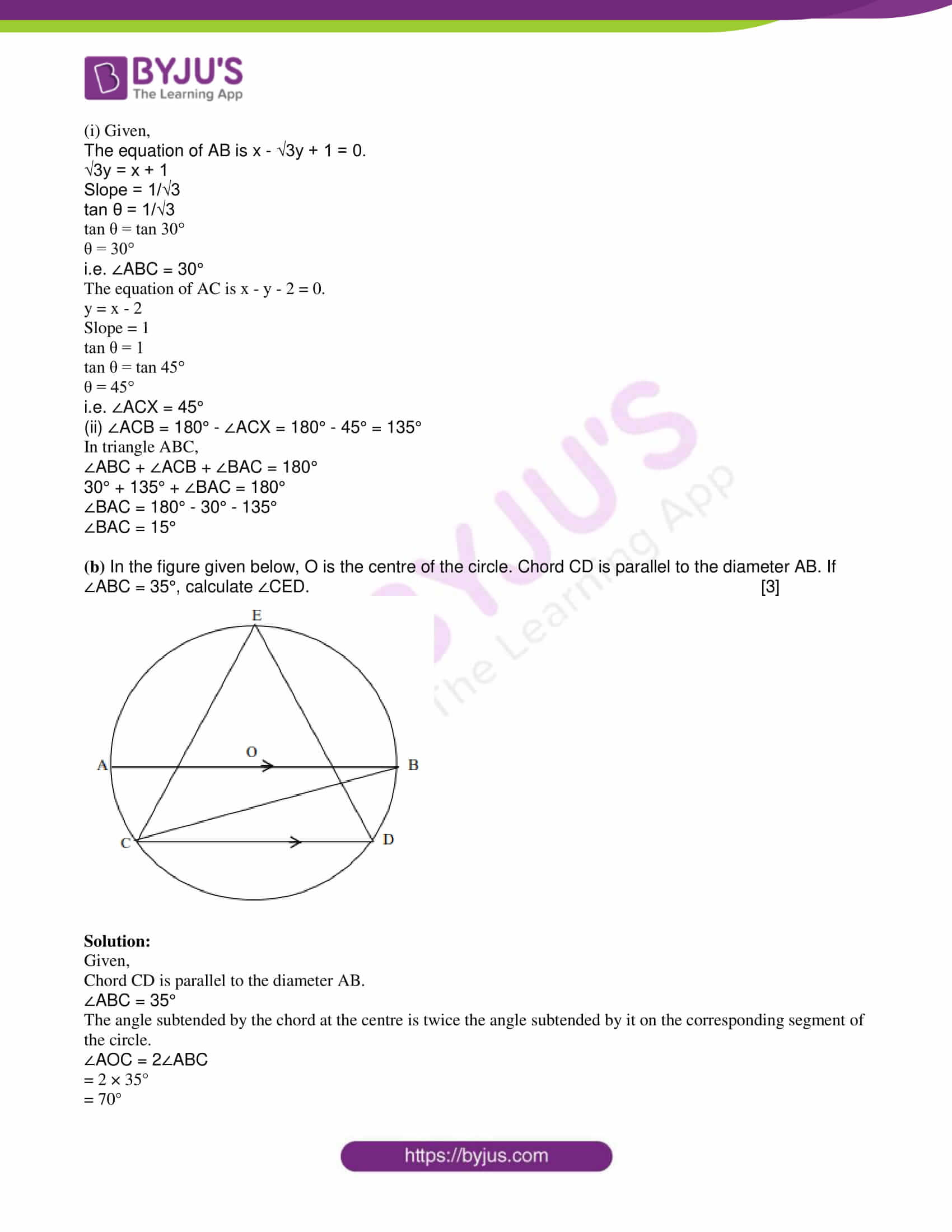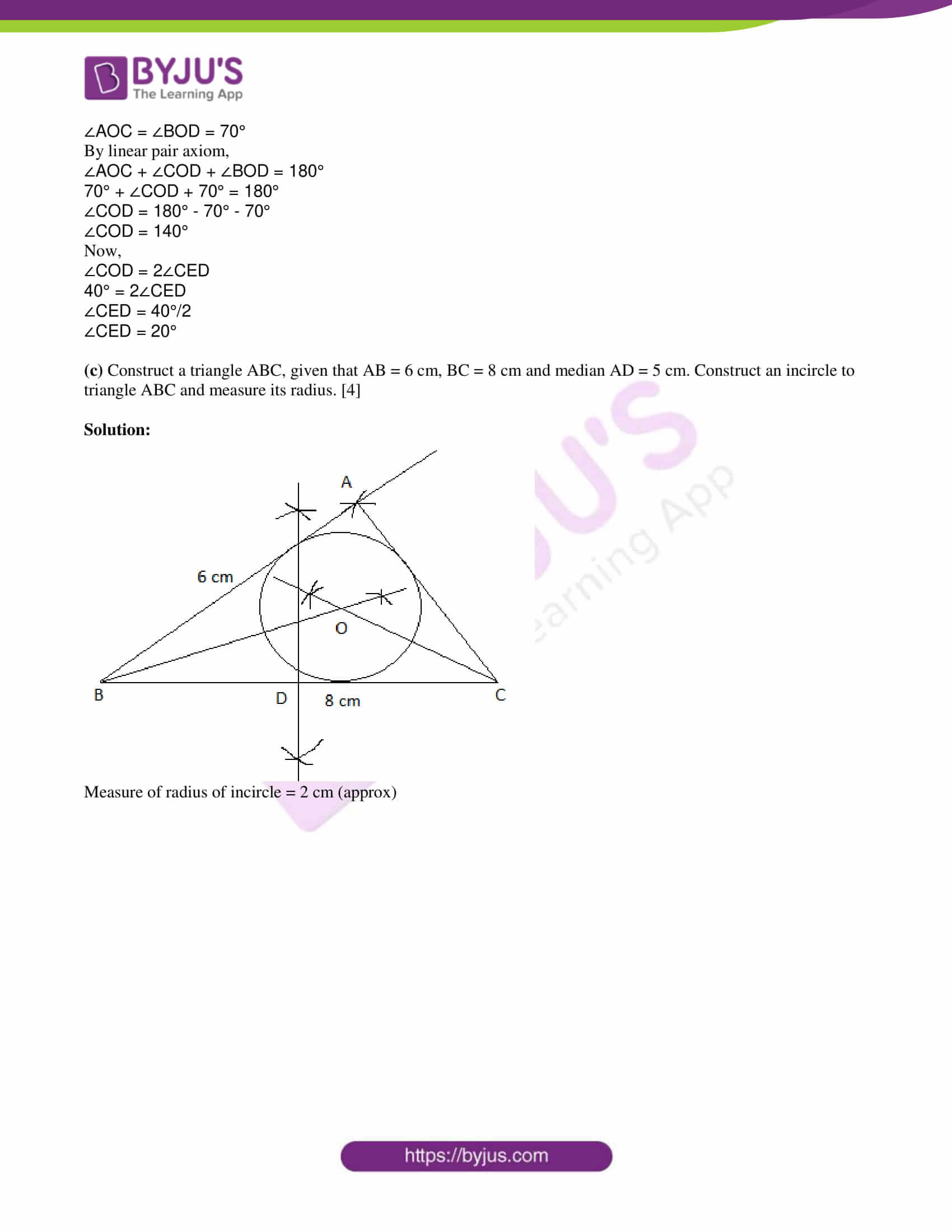SECTION A

Attempt all questions from this section.

Question 1

(a) Find the value of a and b if x – 1 and x – 2 are factors of x3 – ax + b. 

Solution:

Given,

x – 1 and x – 2 are factors of x3 – ax + b.

Let p(x) = x3 – ax + b

p(1) = 0

(1)3 – a(1) + b = 0

1 – a + b = 0

a = b + 1….(i)

p(2) = 0

(2)3 – a(2) + b = 0

8 – 2a + b = 0

8 – 2(b + 1) + b = 0 [From (i)]

8 – 2b – 2 + b = 0

6 – b = 0

b = 6

Substituting b = 6 in (i),

a = 6 + 1 = 7

Therefore, a = 7 and b = 6.

(b) In the figure given below, ABCD is a parallelogram. E is a point on AB. CE intersects the diagonal BD at G and EF is parallel to BC.

If AE : EB = 1 : 2, find

(ii) area of triangle BEF : area of triangle ABD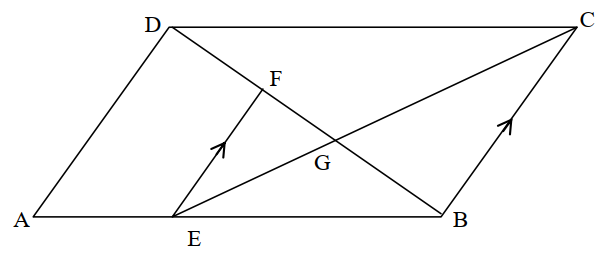Solution:

In triangle BEF and ABD,

∠EBF = ∠ ABD

By AAA similarity criterion,

ΔBEF ~ ΔABD

By BPT,

2/(1 + 2) = EF/AD [given AE/EB = 1/2]

EF : AD = 2 : 3

(ii) The ratio of area of two similar triangles is equal to the ratio of squares of their corresponding sides.

= (2/3)2

= 4/9

(c) On a certain sum of money, the difference between the compound interest for a year, payable half-yearly, and the simple interest for a year is Rs. 16. Find the sum lent out, if the rate of interest in both cases is 8%. 

Solution:

Given,

Rate of interest = 8%

Let P be the sum lent out for interest.

SI = PTR/100

SI = (P × 1 × 8)/100

SI = 2P/25….(i)

CI = P[1 + (R/200)]2 – P (half-yearly payable)

= P[1 + (8/200)]2 – P

= P(208/200)2 – P

= P(43264/40000) – P

CI = 3264P/40000….(ii)

CI – SI = Rs. 16 (given)

(3264P/40000) – (2P/25) = 16

0.0816P – 0.08P = 16

0.0016P = 16

P = 16/0.0016

P = 10000

Therefore, the sum lent out is Rs. 10000.

Question 2

(a) Plot the points A(9, 6) and B(5, 9) on the graph paper. These two points are the vertices of a figure ABCD which is symmetrical about x = 5 and y = 6. Complete the figure on the graph. Write down the geometrical name of the figure. 

Solution: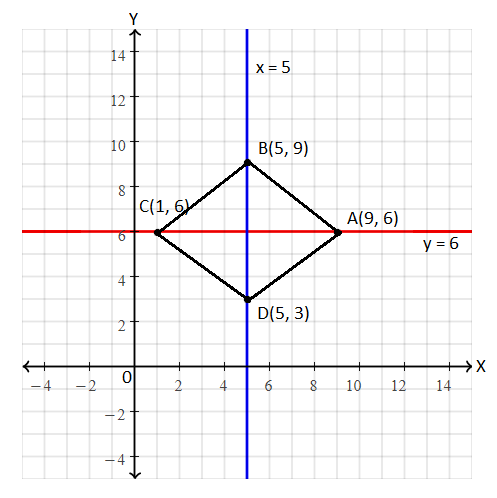Coordinates of C(1, 6) and D(5, 3).

The obtained figure is a rhombus.

(b) In the figure, ABCD is a cyclic quadrilateral in which BC = CD and CF is a tangent to the circle at C. BC is produced to E and ∠DCE = 112°. If O is the centre of the circle, find:

(i) ∠BOC

(ii) ∠DCF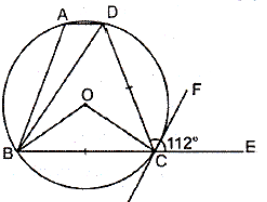Solution:

ABCD is a cyclic quadrilateral in which BC = CD.

CF is a tangent to the circle at C.

BC is produced to E.

∠DCE = 112°

∠ADC = ∠DCE = 112° (alternate angles)

AD || BC and BD is the transversal.

= (½) × 112°

= 56°

∠BOC = 2 ∠BDC (angles of the same segment)

= 2 × 56°

= 112°

(ii) CF is the transversal.

∠DCF = ½ ∠DCE

= (½) × 112°

= 56°

(c) PQRS is a square piece of land of side 56 m. Two semicircular grass-covered lawns are made on two of its opposite sides as shown in the figure. Calculate the area of the uncovered portion.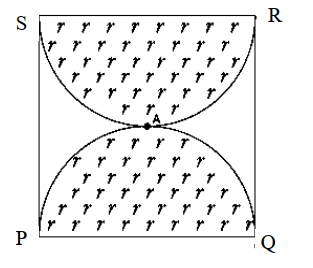Solution:

Given,

Side of square PQRS = a = 56 m

Diameter of semicircle = 56 m

Radius of semicircle = r = 56/2 = 28 m

Area of the uncovered portion = Area of the square – 2 × Area of the semicircle

= a2 – 2 × (πr2/2)

= (56)2 – (22/7) × 28 × 28

= 3136 – 2464

= 672 m2

Question 3

(a)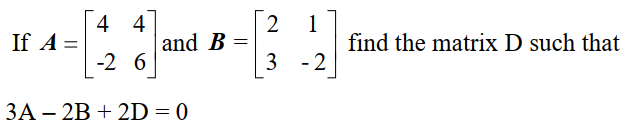Solution:

Given,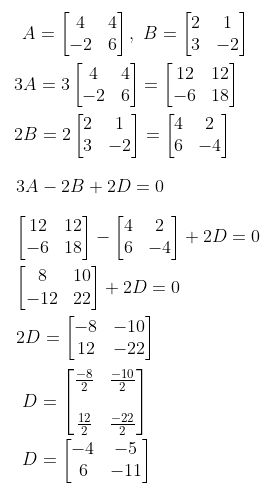(b) A point P(a, b) is reflected in the Y-axis to P1(-3, 1)

Write down the values of a and b.

P11 is the image of P when reflected in the X-axis.

Write down the coordinates of P11.

P111 is the image of P when reflected in the line X = 5.

Write down the coordinates of P111. 

Solution:

Given,

P(a, b) is reflected in the Y-axis to P1(-3, 1).

P11 is the image of P when reflected in the X-axis.

P111 is the image of P when reflected in the line X = 5.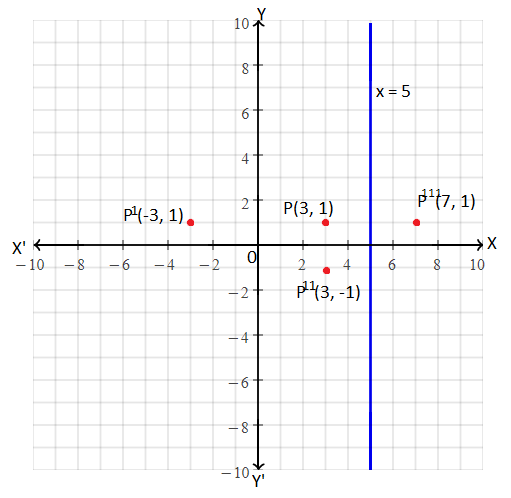Coordinates of P(3, 1)

Thus, a = 3, b = 1

Coordinates of P11 = (3, -1)

Coordinates of P111 = (7, 1)

(c) Given: A = {x : 3 < 2x – 1 < 9, x ∈ R}, B = {x : 11 ≤ 3x + 2 ≤ 23, x ∈ R} where R is the set of real numbers.

(i) Represent A and B on the number line

(ii) On the number line also mark A ⋂ B. 

Solution:

A = {x : 3 < 2x – 1 < 9, x ∈ R}

3 < 2x – 1

3 + 1 < 2x

4 < 2x

x > 4/2

x > 2

And

2x – 1 < 9

2x < 9 + 1

x < 10/2

x < 5

Therefore, 2 < x < 5

A = {3, 4}

B = {x : 11 ≤ 3x + 2 ≤ 23, x ∈ R}

11 ≤ 3x + 2

11 – 2 ≤ 3x

9 ≤ 3x

3 ≤ x

And

3x + 2 ≤ 23

3x ≤ 23 – 2

3x ≤ 21

x ≤ 7

Therefore, 3 ≤ x ≤ 7

B = {3, 4, 5, 6, 7}

A ⋂ B = {3, 4} ⋂ {3, 4, 5, 6, 7} = {3, 4}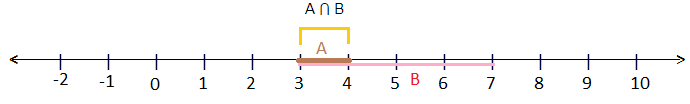Question 4:

(a) Without using a trigonometric table calculate:

4 (sin 32°/ cos 58°) + 5 (tan 48°/ cot 42°) – 8 (sec 72°/ cosec 18°) 

Solution:

4 (sin 32°/ cos 58°) + 5 (tan 48°/ cot 42°) – 8 (sec 72°/ cosec 18°)

= 4 [ sin (90° – 58°)/ cos 58°] + 5 [tan (90° – 42°)/ cot 42°] – 8 [sec (90° – 18°)/ cosec 18°]

= 4 (cos 58°/ cos 58°) + 5 (cot 42°/ cot 42°) – 8 (cosec 18°/ cosec 18°)

= 4(1) + 5(1) – 8(1)

= 4 + 5 – 8

= 1

(b) Mr. Jacob has a two years recurring deposit account in State Bank of India and deposits Rs. 1500 per month. If he receives Rs. 37,875 at the time of maturity, find the rate of interest. 

Solution:

Given,

Principal (P)= Rs. 1500

Time = 2 years

i.e. n = 2 × 12 = 24 months

Let r be the rate of interest.

Amount received at the time of maturity = Total money deposited + Simple Interest

37875 = (P × n) + [{P × n(n + 1)} / (2 × 12)] × (r/100)

37875 = (1500 × 24) + [{1500 × 24 × 25} / 24] × (r/100)

37875 = 36000 + (900000 / 24) × (r/100)

37875 – 36000 = 37500 × (r/100)

r = (1875 × 100)/37500

r = 5

Hence, the rate of interest is 5%.

(c) Calculate the arithmetic mean, correct to one decimal place, for the following frequency distribution of marks obtained in a Geometry test.

 Marks 0 – 10 10 – 20 20 – 30 30 – 40 40 – 50 No. of students 7 13 15 12 3


Solution:

 Marks No. of students (f) Mid-value (x) fx 0 – 10 7 5 35 10 – 20 13 15 195 20 – 30 15 25 375 30 – 40 12 35 420 40 – 50 3 45 135 ∑f = 50 ∑fx = 1160

Mean = ∑fx/ ∑f

= 1160/50

= 23.2

Therefore, the arithmetic mean is 23.2.

SECTION B

Attempt any four questions from this section.

Question 5

(a)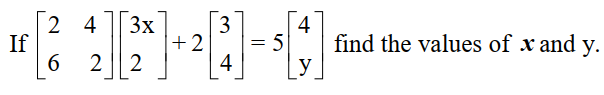Solution:

Given,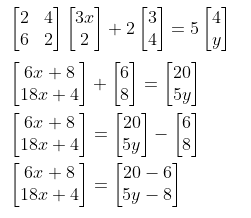6x + 8 = 14

6x = 14 – 8

6x = 6

x = 6/6 = 1

And

18x + 4 = 5y – 8

18(1) + 4 + 8 = 5y

5y = 30

y = 30/5 = 6

Therefore, x = 1 and y = 6.

(b) In the diagram given below, if AF = 21 cm, CE = 30 cm and FB = 7 cm. Find the volume of the figure.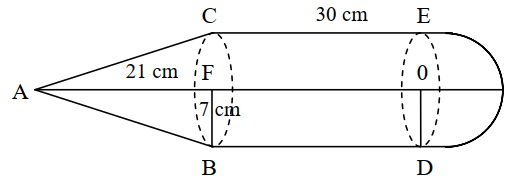Solution:

From the given figure,

Height of the cone = h = 21 cm

Height of the cylinder = H = 30 cm

Volume of the figure = Volume of cone + Volume of cylinder + Volume of hemisphere

= (⅓)πr2h + πr2H + (⅔)πr3

= (1/3) × (22/7) × 7 × 7 × 21 + (22/7) × 7 × 7 × 30 + (2/3) × (22/7) × 7 × 7 × 7

= 22 × 7 × 7 + 22 × 7 × 30 + (2/3) × 22 × 7 × 7

= 1078 + 4620 + 718.67

= 6416.67 cm3

(c) A man bought 200 shares each of face value Rs. 10 at Rs. 12 per share. At the end of the year, the company from which he bought the shares declares a dividend of 15%. Calculate:

(i) the amount of money invested by the man

(ii) the amount of dividend he received

(iii) the percentage return on his outlay. 

Solution:

Number of shares = 200

Nominal value = Rs. 10

Market value = Rs. 12

Dividend = 15%

(i) Investment = Number of shares × Market value

= Rs. 200 × 12

= Rs. 2400

(ii) Dividend = Number of shares × Percentage of dividend × Nominal value

= 200 × 15% × 10

= 200 × (15/100) × 10

= Rs. 300

(iii) Let P be the percentage of return.

Now, Dividend percentage × Nominal value = p% × Market value

15% × 10 = p% × 12

p = (15 × 10)/ 12

p = 25/2

p = 12.5

Hence, the percentage of return on outlay is 12.5%.

Question 6

(a) Solve the following quadratic equation for x and give your answer correct to three significant figures.

2x2 – 4x – 3 = 0 

Solution:

2x2 – 4x – 3 = 0

Dividing by 2,

x2 – 2x – 3/2 = 0

x2 – 2x = 3/2

x2 – 2x + 1 = (3/2) + 1

(x – 1)2 = (5/2)

x – 1 = ± √(5/2)

x = 1 ± √(5/2)

x = 1 ± √2.5

x = 1 ± 1.581

Now,

x = 1 + 1.581, x = 1 – 1.581

x = 2.581, x = -0.581

(b) An integer is chosen at random from 1 to 50. Find the probability that the number is:

(i) divisible by 5

(ii) a perfect cube

(iii) a prime number. 

Solution:

Integers from 1 to 50: {1, 2, 3, 4, 5, …., 49, 50}

Total number of outcomes = n(S) = 50

(i) Number of integers divisible by 5 = {5, 10, 15, 20, 25, 30, 35, 40, 45, 50} = 10

P(getting a number that is divisible by 5) = 10/50 = 1/5

(ii) Perfect cubes = {1, 8, 27} = 3

P(getting a perfect cube number) = 3/50

(iii) Prime numbers = {2, 3,5, 7, 11, 13, 17, 19, 23, 29, 31, 37, 41, 43, 47} = 15

P(getting a prime number) = 15/50

= 3/10

(c) Find x from the following equation using properties of proportion:

(x2 – x + 1)/ (x2 + x + 1) = 14(x – 1)/ 13(x + 1) 

Solution:

Given,

(x2 – x + 1)/ (x2 + x + 1) = 14(x – 1)/ 13(x + 1)

(x2 – x + 1)/ (x2 + x + 1) = (14x – 14)/ (13x + 13)

Using componendo and dividendo,

(x2 – x + 1 + x2 + x + 1) / (x2 – x + 1 – x2 – x – 1) = (14x – 14 + 13x + 13)/ (14x – 14 – 13x – 13)

(2x2 + 2)/ (-2x) = (27x – 1)/ (x – 27)

2(x2 + 1)/ (-2x) = (27x – 1)/ (x – 27)

(x2 + 1)/ (-x) = (27x – 1)/ (x – 27)

⇒ (x2 + 1)(x – 27) = -x(27x – 1)

⇒ x3 – 27x2 + x – 27 = -27x2 + x

⇒ x3 – 27 = 0

⇒ x3 = 27

⇒ x3 = (3)3

⇒ x = 3

Question 7

(a) Bosco wishes to start a 200 m2 rectangular vegetable garden. Since he has only 50 m barbed wire, he fences three sides of the rectangular garden letting his house compound wall act as the fourth side of the fence. Find the dimensions of the garden. 

Solution:

Let x and y be the length and breadth of the garden respectively.

According to the given,

Sum of three sides = 50 m

x + 2y = 50 m

x = 50 – 2y….(i)

Area of the garden = 200 m2 (given)

xy = 200

(50 – 2y)y = 200 [From (i)]

50y – 2y2 = 200

2y2 – 50y + 200 = 0

2(y2 – 25y + 100) = 0

y2 – 25y + 100 = 0

y2 – 20y – 5y + 100 = 0

y(y – 20) – 5(y – 20) = 0

(y – 5)(y – 20) = 0

y = 5, y = 20

When y = 5 m,

x = 50 – 2(5)

= 50 – 10

= 40 m

When y = 20 m,

x = 50 – 2(20)

= 50 – 40

= 10 m

Therefore, the dimensions of the garden or 40 m and 5 m or 10 m and 20 m.

(b) Construct a triangle ABC with AB = 6 cm, BC = 7 cm and ∠ABC = 60°. Locate by construction the point P such that

(i) P is equidistant from B and C

(ii) P is equidistant from AB and BC.

(iii) Measure and record the length of PA. 

Solution: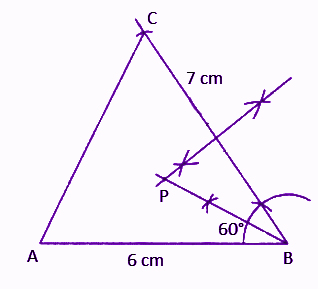The length of the PA is 4.5 cm. (by measure)

(c) Mr. A. Ramchander has an account with the Central Bank of India. The following entries are from his passbook.

 Date Particulars Withdrawal Deposits Balance 05.01.2009 B/F 8000 20.01.2009 To self 2500 04.02.2009 By cash 9000 20.02.2009 By cash 3000 04.03.2009 To self 1000 15.04.2009 By cash 12000

Complete the above page of his passbook and calculate the interest accumulated in four months, January to April at the rate of 3.5% per annum. If the interest is added on 30th April, find his balance on that date. 

Solution:

 Date Particulars Withdrawal Deposits Balance 05.01.2009 B/F 8000 20.01.2009 To self 2500 5500 04.02.2009 By cash 9000 14500 20.02.2009 By cash 3000 17500 04.03.2009 To self 1000 16500 15.04.2009 By cash 12000 28500

Rate of interest = 3.5%

Let us calculate interest on the number of days in four months from 05.01.2009 to 15.04.2009.

Interest = [(8000 × 15 + 5500 × 15 + 14500 × 16 + 17500 × 12 + 16500 × 42) × 3.5]/ 365 × 100

= [(80 × 15 + 55 × 15 + 145 × 16 + 175 × 12 + 165 × 42) × 3.5]/ 365

= (1200 + 825 + 2320 + 2100 + 6930)/ 365

= 13375/ 365

= 36.64

Therefore, interest is Rs. 36.64.

Question 8

(a) Prove that [1/(sec x – tan x)] + [1/ (sec x + tan x)] = 2/cos x. 

Solution:

LHS = [1/(sec x – tan x)] + [1/ (sec x + tan x)]

= [sec x + tan x + sec x – tan x] / [(sec x – tan x) (sec x + tan x)]

= 2 sec x/ (sec2 x – tan2x)

= 2 sec x/ 1

= 2(1/cos x)

= 2/ cos x

= RHS

Hence proved.

(b) In the figure given below, CD is the diameter of the circle which meets the chord AB at P such that AP = BP = 12 cm. If DP = 8 cm, find the radius of the circle.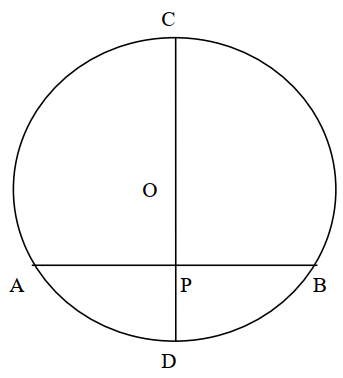Solution:

Given,

AP = BP = 12 cm

DP = 8 cm

Join OA and OB.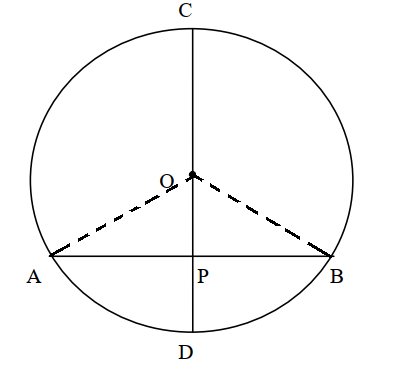Let OP = x

OA = OB = OD = Radius of the circle

OA = OB = OD = (8 + x) cm

In right triangle OPA,

OA2 = OP2 + AP2

(8 + x)2 = x2 + (12)2

64 + x2 + 16x = x2 + 144

16x = 144 – 64

16x = 80

x = 80/16

x = 5

Now,

OD = 8 + 5 = 13 cm

Therefore, the radius of the circle is 13 cm.

(c) Prove that A(2, 1), B(0, 3) and C(-2, 1) are the three vertices of an isosceles right-angled triangle. Hence, find the coordinates of a point D, if ABCD is a square. 

Solution:

Given,

A(2, 1), B(0, 3) and C(-2, 1)

Using distance formula,

AB = √[(0 – 2)2 + (3 – 1)2]

= √(4 + 4)

= √8

BC = √[(-2 – 0)2 + (1 – 3)2]

= √4 + 4)

= √8

AC = √(-2 – 2)2 + (1 – 1)2]

= √(16 + 0)

= √16

Now, AB2 + BC2 = (√8)2 + (√8)2

= 8 + 8

= 16

= AC2

Therefore, ABC is an isosceles right-angled triangle.

Let D(x, y) be the fourth vertex of square ABCD.

Midpoint of AC = Midpoint of BD

[(2 – 2)/2, (1 + 1)/2] = [(0 + x)/2, (3 + y)/2]

(0/2, 2/2) = [x/2, (3 + y)/2]

(0, 1) = [x/2, (3 + y)/2]

x/2 = 0, 3 + y = 2

x = 0, y = -1

Hence, the coordinates of D are (0, -1).

Question 9

(a) A fair die is rolled. Find the probability of getting

(i) 3 on the face of the dice

(ii) an odd number on the face of the dice

(iii) a number greater than 1 on the face of the dice. 

Solution:

Given,

A fair die is rolled.

Sample space = S = {1, 2, 3, 4, 5, 6}

n(S) = 6

(i) Let A be the event of getting 3 on the face of the die.

A = {3}

n(A) = 1

P(A) = n(A)/ n(S)

= 1/3

(ii) Let B be the event of getting an odd number.

B = {1, 3, 5}

n(B) = 3

P(B) = n(B)/ n(S)

= 3/6

= 1/2

(iii) Let C be the event of getting a number greater than 1.

C = {2, 3, 4, 5, 6}

n(C) = 5

P(C) = n(C)/ n(S)

= 5/6

(b) A(4, 2), B(6, 8), and C(8, 4) are the vertices of a triangle ABC. Write down the equation of the median of the triangle through A. 

Solution:

Let AD be the median of triangle ABC.

Given,

A(4, 2), B(6, 8) and C(8, 4)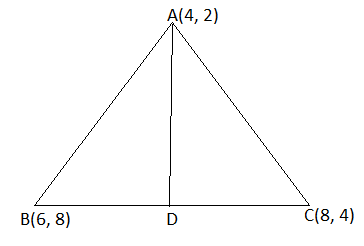D = Midpoint of BC

= [(6 + 8)/2, (8 + 4)/2]

= (14/2, 12/2)

= (7, 6)

Let A(4, 2) = (x1, y1)

D(7, 6) = (x2, y2)

(y – y1)/ (y2 – y1) = (x – x1)/ (x2 – x1)

(y – 2)/ (6 – 2) = (x – 4)/ (7 – 4)

(y – 2)/ 4 = (x – 4)/ 3

3(y – 2) = 4(x – 4)

3y – 6 = 4x – 16

4x – 16 – 3y + 6 = 0

4x – 3y – 10 = 0

Hence, the equation of the median of the triangle through A is 4x – 3y – 10 = 0.

(c) The angle of elevation of an aeroplane from a point P on the ground is 60°. After 12 seconds from the same point P, the angle of elevation of the same plane changes to 30°. If the plane is flying horizontally at a speed of 600√3 km/hr, find the height at which the plane is flying. 

Solution:

Given,

Speed = 600√3 km/hr

Distance = Speed × Time

Distance traveled by aeroplane in 12 seconds = 600√3 × (12/ 60 × 60)

= 2√3 km

Let A be the initial position of an aeroplane.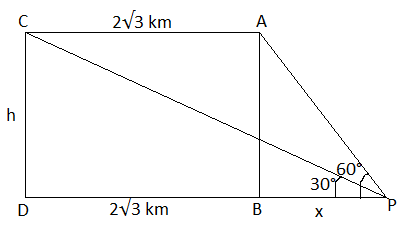AC = BD = 2√3 km

CD = AB = h

In right triangle ABP,

tan 60° = AB/BP

√3 = h/x

⇒ h = √3x….(i)

In right triangle CDP,

tan 30° = CD/DP

1/√3 = h/ (2√3 + x)

2√3 + x = h√3

2√3 + x = (√3x) √3 [From (i)]

2√3 + x = 3x

⇒ 3x – x = 2√3

⇒ 2x = 2√3

⇒ x = √3

Substituting x = √3 in (i),

h = (√3) (√3)

h = 3 km

Hence, the height at which the plane is flying is 3 km.

Question 10

(a) The following table shows the distribution of the heights of a group of students:

 Height (cm) 140 – 145 145 – 150 150 – 155 155 – 160 160 – 165 165 – 170 170 – 175 No.of students 8 12 18 22 26 10 4

Use a graph sheet to draw an Ogive for the distribution.

Use the Ogive to find:

(i) the interquartile range

(ii) the number of students whose height is more than 168 cm

(iii) the number of students whose height is less than 148 cm. 

Solution:

 Height (cm) No.of students (frequency) Cumulative frequency 140 – 145 8 8 145 – 150 12 20 150 – 155 18 38 155 – 160 22 60 160 – 165 26 86 165 – 170 10 96 170 – 175 4 100

Ogive: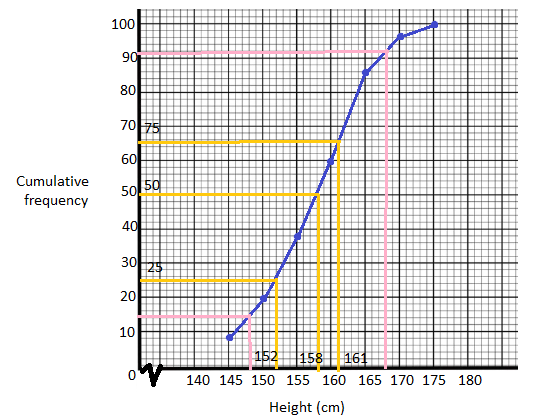(i) Median value = (N/2)th observation = (100/2) = 50th observation = 158

Lower quartile = (N/4)th observation = (100/4) = 25th observation = 152

Upper quartile = (3N/4)th observation = (300/4) = 75th observation = 161

Inter quartile range = Upper quartile – Lower quartile

= 161 – 152

= 9

(ii) The number of students whose height is more than 168 cm = 100 – 92 = 8

(iii) The number of students whose height is less than 148 cm = 14

(b) The manufacturer sold a TV to a wholesaler for Rs. 7000. The wholesaler sold it to a trader at a profit of Rs. 1000. If the trader sold it to the customer at a profit of Rs. 1500, find:

(i) the total VAT (value-added tax) collected by the state government at the rate of 5%.

(ii) the amount that the customer pays for the TV. 

Solution:

Cost price of TV for wholesaler = Rs. 7000

Selling price of TV to a trader from wholesaler = Rs. (7000 + 1000) = Rs. 8000

Selling price of TV from trader = Rs. (8000 + 1500) = Rs. 9500

(i) The total VAT (value added tax) collected by the state government at the rate of 5% = (5/100) × 9500 = Rs. 475

(ii) The amount the customer pays for the TV = Rs. 9500 + Rs. 475https://www.timesnownews.com/education/article/cbse-syllabus-reduced-by-30-for-9th-to-12th-core-concepts-retained-hrd-minister/617823

= Rs. 9975

Question 11:

(a) In the diagram given below, the equation of AB is x – √3y + 1 = 0 and the equation of AC is x – y – 2 = 0.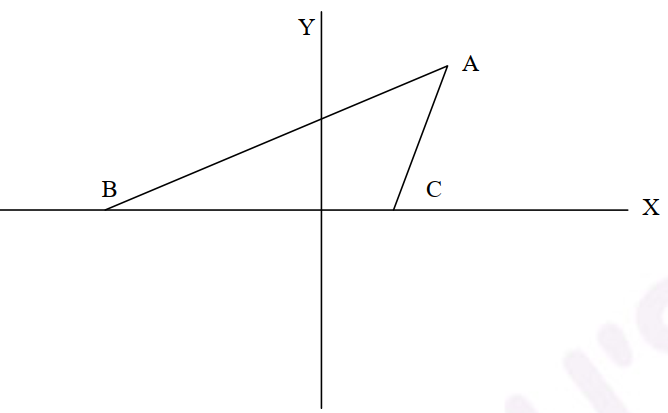(i) Write down the angles that the lines AC and AB make with the positive direction of X-axis.

(ii) Find ∠BAC. 

Solution:

(i) Given,

The equation of AB is x – √3y + 1 = 0.

√3y = x + 1

Slope = 1/√3

tan θ = 1/√3

tan θ = tan 30°

θ = 30°

i.e. ∠ABC = 30°

The equation of AC is x – y – 2 = 0.

y = x – 2

Slope = 1

tan θ = 1

tan θ = tan 45°

θ = 45°

i.e. ∠ACX = 45°

(ii) ∠ACB = 180° – ∠ACX = 180° – 45° = 135°

In triangle ABC,

∠ABC + ∠ACB + ∠BAC = 180°

30° + 135° + ∠BAC = 180°

∠BAC = 180° – 30° – 135°

∠BAC = 15°

(b) In the figure given below, O is the centre of the circle. Chord CD is parallel to the diameter AB. If ∠ABC = 35°, calculate ∠CED.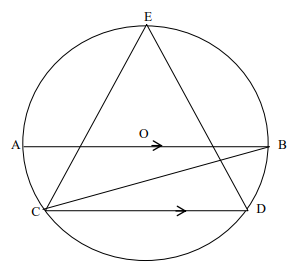Solution:

Given,

Chord CD is parallel to the diameter AB.

∠ABC = 35°

The angle subtended by the chord at the centre is twice the angle subtended by it on the corresponding segment of the circle.

∠AOC = 2∠ABC

= 2 × 35°

= 70°

∠AOC = ∠BOD = 70°

By linear pair axiom,

∠AOC + ∠COD + ∠BOD = 180°

70° + ∠COD + 70° = 180°

∠COD = 180° – 70° – 70°

∠COD = 140°

Now,

∠COD = 2∠CED

40° = 2∠CED

∠CED = 40°/2

∠CED = 20°

(c) Construct a triangle ABC, given that AB = 6 cm, BC = 8 cm and median AD = 5 cm. Construct an incircle to triangle ABC and measure its radius. 

Solution: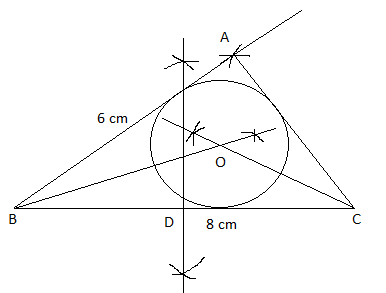Measure of radius of incircle = 2 cm (approx)